# Lower Columbia River Fish Population Indexing 2020

The suggested citation for this analytic report is:

Thorley, J.L. & Hussein, N. (2021) Lower Columbia River Fish Population Indexing 2020. A Poisson Consulting Analysis Appendix. URL: https://www.poissonconsulting.ca/f/392669554.

## Background

In the mid 1990s BC Hydro began operating Hugh L. Keenleyside (HLK) Dam to reduce dewatering of Mountain Whitefish and Rainbow Trout eggs.

The primary goal of the Lower Columbia River Fish Population Indexing program is to answer two key management questions:

What are the abundance, growth rate, survival rate, body condition, age distribution, and spatial distribution of subadult and adult Whitefish, Rainbow Trout, and Walleye in the Lower Columbia River?

What is the effect of inter-annual variability in the Whitefish and Rainbow Trout flow regimes on the abundance, growth rate, survival rate, body condition, and spatial distribution of subadult and adult Whitefish, Rainbow Trout, and Walleye in the Lower Columbia River?

The inter-annual variability in the Whitefish and Rainbow Trout flow regimes was quantified in terms of the percent egg dewatering as greater flow variability is associated with more egg stranding.

## Methods

### Data Preparation

The fish indexing data were provided by Okanagan Nation Alliance and Golder Associates in the form of an Access database. The discharge and temperature data were obtained from the Columbia Basin Hydrological Database maintained by Poisson Consulting. The Rainbow Trout egg dewatering estimates were provided by CLBMON-46 and the Mountain Whitefish egg stranding estimates by Golder Associates (2013).

#### Discharge

Missing hourly discharge values for Hugh-Keenleyside Dam (HLK), Brilliant Dam (BRD) and Birchbank (BIR) were estimated by first leading the BIR values by 2 hours to account for the lag. Values missing at just one of the dams were then estimated assuming $$HLK + BRD = BIR$$. Negative values were set to be zero. Next, missing values spanning $$\leq$$ 28 days were estimated at HLK and BRD based on linear interpolation. Finally any remaining missing values at BIR were set to be $$HLK + BRD$$.

The data were prepared for analysis using R version 4.0.5 .

### Data Analysis

Model parameters were estimated using hierarchical Bayesian methods. The parameters were produced using JAGS and STAN . For additional information on Bayesian estimation the reader is referred to .

The one exception is the length-at-age estimates which were produced using the mixdist R package which implements Maximum Likelihood with Expectation Maximization.

Unless stated otherwise, the Bayesian analyses used weakly informative normal and half-normal prior distributions . The posterior distributions were estimated from 1500 Markov Chain Monte Carlo (MCMC) samples thinned from the second halves of 3 chains . Model convergence was confirmed by ensuring that the potential scale reduction factor $$\hat{R} \leq 1.05$$ and the effective sample size $$\textrm{ESS} \geq 150$$ for each of the monitored parameters .

The parameters are summarised in terms of the point estimate, lower and upper 95% credible limits (CLs) and the surprisal s-value . The estimate is the median (50th percentile) of the MCMC samples while the 95% CLs are the 2.5th and 97.5th percentiles. The s-value can be considered a test of directionality. More specifically it indicates how surprising (in bits) it would be to discover that the true value of the parameter is in the opposite direction to the estimate. An s-value is the Shannon transform (-log to base 2) of the corresponding p-value . A surprisal value of 4.3 bits, which is equivalent to a p-value of 0.05 indicates that the surprise would be equivalent to throwing 4.3 heads in a row. The condition that non-essential explanatory variables have s-values $$\geq$$ 4.3 bits provides a useful model selection heuristic .

Model adequacy was assessed via posterior predictive checks . More specifically, the number of zeros and the first four central moments (mean, variance, skewness and kurtosis) for the deviance residuals were compared to the expected values by simulating new residuals. In this context the s-value indicates how surprising each metric is given the estimated posterior probability distribution for the residual variation.

Where computationally practical, the sensitivity of the parameters to the choice of prior distributions was evaluated by increasing the standard deviations of all normal, half-normal and log-normal priors by an order of magnitude and then using $$\hat{R}$$ to test whether the samples where drawn from the same posterior distribution .

The results are displayed graphically by plotting the modeled relationships between particular variables and the response(s) with the remaining variables held constant. In general, continuous and discrete fixed variables are held constant at their mean and first level values, respectively, while random variables are held constant at their typical values (expected values of the underlying hyperdistributions) . When informative the influence of particular variables is expressed in terms of the effect size (i.e., percent or n-fold change in the response variable) with 95% credible intervals .

The analyses were implemented using R version 4.0.5 and the mbr family of packages.

### Model Descriptions

#### Condition

The expected weight of fish of a given length were estimated from the data using an allometric mass-length model .

$W = \alpha L^{\beta}$

Key assumptions of the condition model include:

• The expected weight is allowed to vary with length and date.
• The expected weight is allowed to vary randomly with year.
• The relationship between weight and length is allowed to vary with date.
• The relationship between weight and length is allowed to vary randomly with year.
• The residual variation in weight is log-normally distributed.

Only previously untagged fish were included in models to avoid potential effects of tagging on body condition. Preliminary analyses indicated that the annual variation in weight was not correlated with the annual variation in the relationship between weight and length.

#### Growth

Annual growth of fish were estimated from the inter-annual recaptures using the Fabens method for estimating the von Bertalanffy growth curve . This curve is based on the premise that:

$\frac{\text{d}L}{\text{d}t} = k (L_{\infty} - L)$

where $$L$$ is the length of the individual, $$k$$ is the growth coefficient and $$L_{\infty}$$ is the maximum length.

Integrating the above equation gives:

$L_t = L_{\infty} (1 - e^{-k(t - t_0)})$

where $$L_t$$ is the length at time $$t$$ and $$t_0$$ is the time at which the individual would have had zero length.

The Fabens form allows

$L_r = L_c + (L_{\infty} - L_c) (1 - e^{-kT})$

where $$L_r$$ is the length at recapture, $$L_c$$ is the length at capture and $$T$$ is the time between capture and recapture.

Key assumptions of the growth model include:

• The mean maximum length $$L_{\infty}$$ is constant.
• The growth coefficient $$k$$ is allowed to vary randomly with year.
• The residual variation in growth is normally distributed.

The growth model was only fitted to Walleye with a fork length at release less than 450 mm.

#### Movement

The extent to which sites are closed, i.e., fish remain at the same site between sessions, was evaluated with a logistic ANCOVA . The model estimates the probability that intra-annual recaptures were caught at the same site versus a different one. Key assumptions of the site fidelity model include:

• The expected site fidelity is allowed to vary with fish length.
• Observed site fidelity is Bernoulli distributed.

Length as a second-order polynomial was not found to be a significant predictor for site fidelity.

The estimated probability of being caught at the same site versus a different site was then converted into the site fidelity by assuming that those fish which were recaught at a different site represented just 32 % of those that left the site. The correction factor corresponds to the proportion of the river bank that belongs to index sites.

#### Length-At-Age

The expected length-at-age of Mountain Whitefish and Rainbow Trout were estimated from annual length-frequency distributions using a finite mixture distribution model

There were assumed to be three distinguishable normally-distributed age-classes for Mountain Whitefish (Age-0, Age-1, Age-2 and Age-3+) two for Rainbow Trout (Age-0, Age-1, Age-2+). Initially the model was fitted to the data from all years combined. The model was then fitted to the data for each year separately with the initial values set to be the estimates from the combined values. The only constraints were that the standard deviations of the MW age-classes were identical in the combined analysis and fixed at the initial values in the individual years.

Rainbow Trout and Mountain Whitefish were categorized as Fry (Age-0), Juvenile (Age-1) and Adult (Age-2+) based on their length-based ages. All Walleye were considered to be Adults.

#### Survival

The annual adult survival rate was estimated by fitting a Cormack-Jolly-Seber model to inter-annual recaptures of adults.

Key assumptions of the survival model include:

• Survival varies randomly with year.
• The encounter probability for adults is allowed to vary with the total bank length sampled.

Preliminary analysis indicated that only including visits to index sites did not substantially change the results.

#### Observer Length Correction

The annual bias (inaccuracy) and error (imprecision) in observer’s fish length estimates were quantified from the divergence of the length distribution of their observed fish from the length distribution of the measured fish. More specifically, the length correction that minimised the Jensen-Shannon divergence (Lin 1991) between the two distributions provided a measure of the inaccuracy while the minimum divergence (the Jensen-Shannon divergence was calculated with log to base 2 which means it lies between 0 and 1) provided a measure of the imprecision.

#### Capture Efficiency

The probability of capture was estimated using a recapture-based binomial model .

Key assumptions of the capture efficiency model include:

• The capture probability varies randomly by session within year.
• The probability of a marked fish remaining at a site is the estimated site fidelity.
• The number of recaptures is described by a binomial distribution.

Preliminary analyses indicated that the direction of effect of the frequency of the electrofishing current (30, 60 or 120 Hz) was uncertain.

#### Abundance

The abundance was estimated from the catch and bias-corrected observer count data using an overdispersed Poisson model .

Key assumptions of the abundance model include:

• The fish density varies randomly with site, year and site within year.
• The capture efficiency at a typical fish density is the point estimate for a typical session from the capture efficiency model.
• The count efficiency varies from the capture efficiency.
• The capture efficiency (but not the count efficiency) varies with density.
• The overdispersion varies by visit type (count or catch).
• The catches and counts are described by a gamma-Poisson distribution.
##### Distribution

The distribution was calculated in terms of the Shannon index of evenness in each year for each species and life-stage. The index was calculated using the following formula where $$S$$ is the number of sites and $$p_i$$ is the proportion of the total density belonging to the ith site

$E = \frac{-\sum p_i \log(p_i)}{\log(S)}$

#### Survival (Abundance-based)

The subadult ($$S_t$$) and adult ($$A_t$$) abundance estimates were used to calculate the subadult and adult survival ($$\phi_t$$) in year $$t$$ based on the relationship

$\phi_t = \frac{A_t}{S_{t-1} + A_{t-1}}$

#### Weight

The weight ($$W_t$$) in year $$t$$ was estimated from the expected adult length using the condition model.

#### Fecundity

##### Mountain Whitefish

The fecundity-weight relationship for Mountain Whitefish was estimated from data collected by for the Madison River, Montana. The data were analysed using an allometric model of the form

$F = \alpha W^{\beta}$

Key assumptions of the fecundity model include:

• The residual variation in fecundity is log-normally distributed.
##### Rainbow Trout

Following the fecundity ($$F_t$$) in year $$t$$ of an adult female Rainbow Trout was calculated from the expected weight ($$W_t$$) in grams using the equation:

$F_t = 3.8 \cdot W_t^{0.9}$

#### Egg Deposition

The total egg deposition ($$E_t$$) in year $$t$$ was calculated according to the equation $E_t = F_t * \frac{A_t}{2}$

#### Stock-Recruitment

The relationship between the total number of eggs deposited ($$E_t$$) and the resultant number of subadults (age-1 recruits) ($$S_{t+1}$$) was estimated using a Beverton-Holt stock-recruitment model :

$S_{t+1} = \frac{\alpha \cdot E_t}{1 + \beta \cdot E_t}$

where $$\alpha$$ is the egg to age-1 survival at low density and $$\beta$$ is the density-dependence.

Key assumptions of the stock-recruitment model include:

• The egg to recruit survival at low density ($$\alpha$$) was likely less than 1% (the prior distribution for $$\alpha$$ was a zero truncated normal with standard deviation of 0.005.
• The residual variation in the number of recruits is log-normally distributed.

The expected egg survival for a given egg deposition is $$S / E_t$$ which is given by the equation

$\phi_E = \frac{\alpha}{1 + \beta * E}$

#### Age-Ratios

The proportion of Age-1 Mountain Whitefish $$r^1_t$$ from a given spawn year $$t$$ is calculated from the relative abundance of Age-1 & Age-2 fish $$N^1_t$$ & $$N^2_t$$ respectively, which were lead or lagged so that all values were with respect to the spawn year:

$r^1_t = \frac{N^1_{t+2}}{N^1_{t+2} + N^2_{t+2}}$

The relative abundances of Age-1 and Age-2 fish were taken from the proportions of each age-class in the length-at-age analysis.

As the number of Age-2 fish might be expected to be influenced by the percentage egg loss $$Q_t$$ three years prior, the predictor variable $$\Pi_t$$ used is:

$\Pi_t = \textrm{log}(Q_t/Q_{t-1})$

The ratio was logged to ensure it was symmetrical about zero .

The relationship between $$r^1_t$$ and $$\Pi_t$$ was estimated using a Bayesian regression loss model.

Key assumptions of the final model include:

• The log odds of the proportion of Age-1 fish varies with the log of the ratio of the percent egg losses.
• The residual variation is normally distributed.

The relationship between percent dewatering and subsequent recruitment is expected to depend on stock abundance which might be changing over the course of the study. Consequently, preliminary analyses allowed the slope of the regression line to change by year. However, year was not a significant predictor and was therefore removed from the final model. The effect of dewatering on Mountain Whitefish abundance was expressed in terms of the predicted percent change in Age-1 Mountain Whitefish abundance by egg loss in the spawn year relative to 10% egg loss in the spawn year. The egg loss in the previous year was fixed at 10%. The percent change could not be calculated relative to 0% in the spawn or previous year as $$\Pi_t$$ is undefined in either case.

### Model Templates

#### Condition

 data {
int nYear;
int nObs;

vector[nObs] Length;
vector[nObs] Weight;
vector[nObs] Dayte;
int Year[nObs];

parameters {
real bWeight;
real bWeightLength;
real bWeightDayte;
real bWeightLengthDayte;
real<lower=0> sWeightYear;
real<lower=0> sWeightLengthYear;

vector[nYear] bWeightYear;
vector[nYear] bWeightLengthYear;
real<lower=0> sWeight;

model {

vector[nObs] eWeight;

bWeight ~ normal(5, 4);
bWeightLength ~ normal(3, 1);

bWeightDayte ~ normal(0, 1);
bWeightLengthDayte ~ normal(0, 1);

sWeightYear ~ normal(0, 1);
sWeightLengthYear ~ normal(0, 1);

for (i in 1:nYear) {
bWeightYear[i] ~ normal(0, sWeightYear);
bWeightLengthYear[i] ~ normal(0, sWeightLengthYear);
}

sWeight ~ normal(0, 5);
for(i in 1:nObs) {
eWeight[i] = bWeight + bWeightDayte * Dayte[i] + bWeightYear[Year[i]] + (bWeightLength + bWeightLengthDayte * Dayte[i] + bWeightLengthYear[Year[i]]) * Length[i];
Weight[i] ~ lognormal(eWeight[i], sWeight);
}

Block 1.

#### Growth

.model {
bK ~ dnorm (0, 5^-2)
sKYear ~ dnorm(0, 2^-2) T(0,)

for (i in 1:nYear) {
bKYear[i] ~ dnorm(0, sKYear^-2)
log(eK[i]) <- bK + bKYear[i]
}

bLinf ~ dunif(200, 1000)
sGrowth ~ dnorm(0, 25^-2) T(0,)
for (i in 1:length(Year)) {
eGrowth[i] <- max(0, (bLinf - LengthAtRelease[i]) * (1 - exp(-sum(eK[Year[i]:(Year[i] + dYears[i] - 1)]))))
Growth[i] ~ dnorm(eGrowth[i], sGrowth^-2)
}

Block 2.

#### Movement

.model {

bFidelity ~ dnorm(0, 1^-2)
bLength ~ dnorm(0, 1^-2)

for (i in 1:length(Fidelity)) {
logit(eFidelity[i]) <- bFidelity + bLength * Length[i]
Fidelity[i] ~ dbern(eFidelity[i])
}

Block 3.

#### Survival

.model{
bEfficiency ~ dnorm(0, 4^-2)
bEfficiencySampledLength ~ dnorm(0, 4^-2)

bSurvival ~ dnorm(0, 4^-2)

sSurvivalYear ~ dnorm(0, 4^-2) T(0,)
for(i in 1:nYear) {
bSurvivalYear[i] ~ dnorm(0, sSurvivalYear^-2)
}

for(i in 1:(nYear-1)) {
logit(eEfficiency[i]) <- bEfficiency + bEfficiencySampledLength * SampledLength[i]
logit(eSurvival[i]) <- bSurvival + bSurvivalYear[i]

eProbability[i,i] <- eSurvival[i] * eEfficiency[i]
for(j in (i+1):(nYear-1)) {
eProbability[i,j] <- prod(eSurvival[i:j]) * prod(1-eEfficiency[i:(j-1)]) * eEfficiency[j]
}
for(j in 1:(i-1)) {
eProbability[i,j] <- 0
}
}
for(i in 1:(nYear-1)) {
eProbability[i,nYear] <- 1 - sum(eProbability[i,1:(nYear-1)])
}

for(i in 1:(nYear - 1)) {
Marray[i, 1:nYear] ~ dmulti(eProbability[i,], Released[i])
}

Block 4.

#### Capture Efficiency

.model {

bEfficiency ~ dnorm(-4, 2^-2)

sEfficiencySessionAnnual ~ dnorm(0, 1^-2) T(0,)
for (i in 1:nSession) {
for (j in 1:nAnnual) {
bEfficiencySessionAnnual[i, j] ~ dnorm(0, sEfficiencySessionAnnual^-2)
}
}

for (i in 1:length(Recaptures)) {

logit(eEfficiency[i]) <- bEfficiency + bEfficiencySessionAnnual[Session[i], Annual[i]]

eFidelity[i] ~ dnorm(Fidelity[i], FidelitySD[i]^-2) T(FidelityLower[i], FidelityUpper[i])
Recaptures[i] ~ dbin(eEfficiency[i] * eFidelity[i], Tagged[i])
}

Block 5.

#### Abundance

.model {
bDensity ~ dnorm(5, 4^-2)

sDensityAnnual ~ dnorm(0, 1^-2) T(0,)
for (i in 1:nAnnual) {
bDensityAnnual[i] ~ dnorm(0, sDensityAnnual^-2)
}

sDensitySite ~ dnorm(0, 1^-2) T(0,)
sDensitySiteAnnual ~ dnorm(0, 1^-2) T(0,)
for (i in 1:nSite) {
bDensitySite[i] ~ dnorm(0, sDensitySite^-2)
for (j in 1:nAnnual) {
bDensitySiteAnnual[i, j] ~ dnorm(0, sDensitySiteAnnual^-2)
}
}

bEfficiencyVisitType <- 0
bEfficiencyVisitTypeDensity ~ dnorm(0, 2^-2)
for (i in 2:nVisitType) {
bEfficiencyVisitType[i] ~ dnorm(0, 2^-2)
bEfficiencyVisitTypeDensity[i] <- 0
}

sDispersion ~ dnorm(0, 1^-2)
sDispersionVisitType <- 0
for(i in 2:nVisitType) {
sDispersionVisitType[i] ~ dnorm(0, 2^-2)
}

for (i in 1:length(Fish)) {
log(eDensity[i]) <- bDensity + bDensitySite[Site[i]] + bDensityAnnual[Annual[i]] + bDensitySiteAnnual[Site[i],Annual[i]]

eAbundance[i] <- eDensity[i] * SiteLength[i]

logit(eEfficiency[i]) <- logit(Efficiency[i]) + bEfficiencyVisitType[VisitType[i]] + bEfficiencyVisitTypeDensity[VisitType[i]] * (eDensity[i] - exp(bDensity + sDensityAnnual^2/2 + sDensitySite^2/2 + sDensitySiteAnnual^2/2))

log(esDispersion[i]) <- sDispersion + sDispersionVisitType[VisitType[i]]

eDispersion[i] ~ dgamma(esDispersion[i]^-2 + 0.1, esDispersion[i]^-2 + 0.1)
eFish[i] <- eAbundance[i] * ProportionSampled[i] * eEfficiency[i]
Fish[i] ~ dpois(eFish[i] * eDispersion[i])
}

Block 6.

#### Fecundity

model {
bFecundity ~ dnorm(0, 5^-2)
bFecundityWeight ~ dnorm(1, 1^-2) T(0,)

sFecundity ~ dnorm(0, 1^-2) T(0,)
for(i in 1:length(Weight)) {
eFecundity[i] = bFecundity + bFecundityWeight * log(Weight[i])
Fecundity[i] ~ dlnorm(eFecundity[i], sFecundity^-2)
}

Block 7.

#### Stock-Recruitment

.model {
bAlpha ~ dnorm(0, 0.003^-2) T(0,)
bBeta ~ dnorm(0, 0.007^-2) T(0, )
bEggLoss ~ dnorm(0, 100^-2)

sRecruits ~ dnorm(0, 1^-2) T(0,)
for(i in 1:length(Recruits)){
log(eRecruits[i]) <- log(bAlpha * Eggs[i] / (1 + bBeta * Eggs[i])) + bEggLoss * EggLoss[i]
Recruits[i] ~ dlnorm(log(eRecruits[i]), sRecruits^-2)
}

Block 8.

#### Age-Ratios

.model{
bProbAge1 ~ dnorm(0, 1^-2)
bProbAge1Loss ~ dnorm(0, 1^-2)

sProbAge1 ~ dnorm(0, 1^-2) T(0,)
for(i in 1:length(Age1Prop)){
eAge1Prop[i] <- bProbAge1 + bProbAge1Loss * LossLogRatio[i]
Age1Prop[i] ~ dnorm(eAge1Prop[i], sProbAge1^-2)
}

Block 9.

## Results

### Tables

#### Condition

Table 1. Parameter descriptions.

Parameter Description
bWeight Intercept of log(eWeight)
bWeightDayte Effect of Dayte on bWeight
bWeightLength Intercept of effect of Length on bWeight
bWeightLengthDayte Effect of Dayte on bWeightLength
bWeightLengthYear[i] Effect of ith Year on bWeightLength
bWeightYear[i] Effect of ith Year on bWeight
Dayte[i] Standardised day of year ith fish was captured
eWeight[i] Expected Weight of ith fish
Length[i] Log-transformed and centered fork length of ith fish
sWeight Log standard deviation of residual variation in log(Weight)
sWeightLengthYear Log standard deviation of bWeightLengthYear
sWeightYear Log standard deviation of bWeightYear
Weight[i] Recorded weight of ith fish
Year[i] Year ith fish was captured
##### Mountain Whitefish

Table 2. Model coefficients.

term estimate lower upper svalue
bWeight 5.4729178 5.4544136 5.4920389 10.55171
bWeightDayte -0.0197915 -0.0231447 -0.0163968 10.55171
bWeightLength 3.1611042 3.1208279 3.2017754 10.55171
bWeightLengthDayte -0.0147155 -0.0237248 -0.0054150 8.22978
sWeight 0.1471742 0.1455375 0.1488543 10.55171
sWeightLengthYear 0.1015518 0.0722011 0.1491862 10.55171
sWeightYear 0.0463121 0.0347305 0.0657113 10.55171

Table 3. Model summary.

n K nchains niters nthin ess rhat converged
15354 7 3 500 2 254 1.022 TRUE

Table 4. Model posterior predictive checks.

moment observed median lower upper svalue
zeros 0.0000000 0.0000000 0.0000000 0.0000000 0.0000000
mean 0.0005386 0.0005195 -0.0209618 0.0231632 0.0057785
variance 1.9925704 2.0010932 1.9583630 2.0426788 0.5307283
skewness -0.6146788 -0.0001310 -0.0382044 0.0357064 10.5517083
kurtosis 1.8300308 -0.0030373 -0.0772380 0.0749497 10.5517083

Table 5. Model sensitivity.

n K nchains niters rhat_1 rhat_2 rhat_all converged
15354 7 3 500 1.022 1.018 1.017 TRUE
##### Rainbow Trout

Table 6. Model coefficients.

term estimate lower upper svalue
bWeight 6.0183556 6.0074755 6.0290114 10.551708
bWeightDayte -0.0037957 -0.0061465 -0.0014348 8.966746
bWeightLength 2.9230074 2.8982078 2.9474953 10.551708
bWeightLengthDayte 0.0386003 0.0312429 0.0456394 10.551708
sWeight 0.1016007 0.1005167 0.1028162 10.551708
sWeightLengthYear 0.0525387 0.0376323 0.0767291 10.551708
sWeightYear 0.0255990 0.0193251 0.0358481 10.551708

Table 7. Model summary.

n K nchains niters nthin ess rhat converged
16316 7 3 500 2 366 1.009 TRUE

Table 8. Model posterior predictive checks.

moment observed median lower upper svalue
zeros 0.0000000 0.0000000 0.0000000 0.0000000 0.0000000
mean -0.0001263 -0.0001211 -0.0221990 0.0218578 0.0000000
variance 1.9938608 1.9995316 1.9572274 2.0446986 0.3337506
skewness -0.6974188 0.0002515 -0.0370773 0.0369357 10.5517083
kurtosis 2.5338159 -0.0000042 -0.0737716 0.0770711 10.5517083

Table 9. Model sensitivity.

n K nchains niters rhat_1 rhat_2 rhat_all converged
16316 7 3 500 1.009 1.008 1.008 TRUE
##### Walleye

Table 10. Model coefficients.

term estimate lower upper svalue
bWeight 6.2820584 6.2676127 6.2972647 10.551708
bWeightDayte 0.0157745 0.0130837 0.0183718 10.551708
bWeightLength 3.2310820 3.1958506 3.2664993 10.551708
bWeightLengthDayte -0.0069059 -0.0224187 0.0092320 1.291965
sWeight 0.0924966 0.0911273 0.0937570 10.551708
sWeightLengthYear 0.0756821 0.0533414 0.1083093 10.551708
sWeightYear 0.0347314 0.0260692 0.0490480 10.551708

Table 11. Model summary.

n K nchains niters nthin ess rhat converged
9980 7 3 500 2 316 1.007 TRUE

Table 12. Model posterior predictive checks.

moment observed median lower upper svalue
zeros 0.0000000 0.0000000 0.0000000 0.0000000 0.0000000
mean -0.0000885 0.0002842 -0.0283217 0.0270787 0.0291267
variance 1.9904989 1.9993769 1.9465356 2.0532346 0.3780311
skewness -0.0417045 0.0013398 -0.0488154 0.0495591 3.4121569
kurtosis 1.0661194 -0.0010468 -0.0945997 0.1006134 10.5517083

Table 13. Model sensitivity.

n K nchains niters rhat_1 rhat_2 rhat_all converged
9980 7 3 500 1.007 1.011 1.008 TRUE

#### Growth

Table 14. Parameter descriptions.

Parameter Description
bK Intercept of log(eK)
bKYear[i] Effect of ith Year on bK
bLinf Mean maximum length
dYears[i] Years between release and recapture of ith recapture
eGrowth Expected Growth between release and recapture
eK[i] Expected von Bertalanffy growth coefficient from i-1th to ith year
Growth[i] Observed growth between release and recapture of ith recapture
LengthAtRelease[i] Length at previous release of ith recapture
sGrowth Log standard deviation of residual variation in Growth
sKYear Log standard deviation of bKYear
Year[i] Release year of ith recapture
##### Mountain Whitefish

Table 15. Model coefficients.

term estimate lower upper svalue
bK -0.9411320 -1.1641675 -0.7400515 10.55171
bLinf 395.2888775 389.0096367 400.9345856 10.55171
sGrowth 11.3642806 10.4453562 12.3896129 10.55171
sKYear 0.3613933 0.2284925 0.5799047 10.55171

Table 16. Model summary.

n K nchains niters nthin ess rhat converged
278 4 3 500 50 1020 1.006 TRUE

Table 17. Model posterior predictive checks.

moment observed median lower upper svalue
zeros 4.0000000 4.0000000 4.0000000 4.0000000 10.551708
mean 0.0810325 0.0024308 -0.1648646 0.1663886 1.389317
variance 1.8674479 1.9990016 1.6770072 2.3559267 1.218553
skewness -0.1937519 0.0012519 -0.2828312 0.2793555 2.534900
kurtosis 0.5917619 -0.0601923 -0.4945140 0.6153120 4.012550

Table 18. Model sensitivity.

n K nchains niters rhat_1 rhat_2 rhat_all converged
278 4 3 500 1.006 1.005 1.002 TRUE
##### Rainbow Trout

Table 19. Model coefficients.

term estimate lower upper svalue
bK -0.1565379 -0.3143349 0.0033852 4.247928
bLinf 482.8403628 477.8917278 488.1238806 10.551708
sGrowth 29.7348723 28.6493707 31.0052923 10.551708
sKYear 0.2989984 0.2164984 0.4510284 10.551708

Table 20. Model summary.

n K nchains niters nthin ess rhat converged
1343 4 3 500 50 753 1.006 TRUE

Table 21. Model posterior predictive checks.

moment observed median lower upper svalue
zeros 2.0000000 2.0000000 2.0000000 2.0000000 10.5517083
mean 0.0145462 -0.0001517 -0.0734551 0.0747320 0.5059486
variance 1.9714761 1.9996928 1.8534680 2.1502949 0.4471095
skewness 0.2746833 -0.0026533 -0.1283162 0.1299573 10.5517083
kurtosis 0.7049691 -0.0175134 -0.2327890 0.2471415 10.5517083

Table 22. Model sensitivity.

n K nchains niters rhat_1 rhat_2 rhat_all converged
1343 4 3 500 1.006 1.004 1.004 TRUE
##### Walleye

Table 23. Model coefficients.

term estimate lower upper svalue
bK -2.5355083 -3.0570148 -2.0651447 10.55171
bLinf 743.6815880 623.7372575 963.1322716 10.55171
sGrowth 17.8484294 16.4112282 19.5333356 10.55171
sKYear 0.3237749 0.2030559 0.5174272 10.55171

Table 24. Model summary.

n K nchains niters nthin ess rhat converged
272 4 3 500 50 211 1.011 TRUE

Table 25. Model posterior predictive checks.

moment observed median lower upper svalue
zeros 0.0000000 0.0000000 0.0000000 0.0000000 0.000000
mean 0.0554996 0.0004975 -0.1585835 0.1545668 1.086142
variance 1.8761289 1.9934275 1.6775245 2.3604741 1.102560
skewness 0.2016027 -0.0005644 -0.2725528 0.2885685 2.580165
kurtosis 1.5982252 -0.0605098 -0.5023125 0.6397870 10.551708

Table 26. Model sensitivity.

n K nchains niters rhat_1 rhat_2 rhat_all converged
272 4 3 500 1.011 1.01 1.009 TRUE

#### Movement

Table 27. Parameter descriptions.

Parameter Description
bFidelity Intercept of logit(eFidelity)
bLength Effect of length on logit(eFidelity)
eFidelity[i] Expected site fidelity of ith recapture
Fidelity[i] Whether the ith recapture was encountered at the same site as the previous encounter
Length[i] Length at previous encounter of ith recapture
##### Mountain Whitefish

Table 28. Model coefficients.

term estimate lower upper svalue
bFidelity -0.1564636 -0.5196967 0.2188765 1.2686199
bLength -0.1108706 -0.4588498 0.2432566 0.8917124

Table 29. Model summary.

n K nchains niters nthin ess rhat converged
119 2 3 500 1 852 1 TRUE

Table 30. Model posterior predictive checks.

moment observed median lower upper svalue
zeros 0.0000000 0.0000000 0.0000000 0.0000000 0.0000000
mean -0.0255215 -0.0242748 -0.2450717 0.1937379 0.0096437
variance 1.3880966 1.3734742 1.2393342 1.4124523 0.7172372
skewness 0.1519735 0.1516939 -0.3580415 0.6574335 0.0193523
kurtosis -1.9705203 -1.9326556 -1.9973913 -1.5143380 0.8810520

Table 31. Model sensitivity.

n K nchains niters rhat_1 rhat_2 rhat_all converged
119 2 3 500 1 1.003 1.002 TRUE
##### Rainbow Trout

Table 32. Model coefficients.

term estimate lower upper svalue
bFidelity 0.7548157 0.6053048 0.9113941 10.55171
bLength -0.3263096 -0.4809025 -0.1778207 10.55171

Table 33. Model summary.

n K nchains niters nthin ess rhat converged
813 2 3 500 1 867 1.002 TRUE

Table 34. Model posterior predictive checks.

moment observed median lower upper svalue
zeros 0.0000000 0.0000000 0.0000000 0.0000000 0.0000000
mean 0.1116825 0.1099431 0.0316024 0.1879082 0.0548545
variance 1.2236999 1.2243711 1.1249413 1.3018679 0.0174054
skewness -0.7440932 -0.7463783 -0.9720361 -0.5221097 0.0213019
kurtosis -1.3852954 -1.3760060 -1.6620303 -0.9604425 0.0548545

Table 35. Model sensitivity.

n K nchains niters rhat_1 rhat_2 rhat_all converged
813 2 3 500 1.002 1.002 1.003 TRUE
##### Walleye

Table 36. Model coefficients.

term estimate lower upper svalue
bFidelity 0.6727876 0.4061552 0.9524477 10.5517083
bLength -0.0735188 -0.3447724 0.1749418 0.8085569

Table 37. Model summary.

n K nchains niters nthin ess rhat converged
229 2 3 500 1 718 1.001 TRUE

Table 38. Model posterior predictive checks.

moment observed median lower upper svalue
zeros 0.0000000 0.0000000 0.0000000 0.0000000 0.0000000
mean 0.1079104 0.1016668 -0.0557015 0.2507811 0.1035920
variance 1.2693950 1.2709937 1.0796669 1.3788087 0.0310896
skewness -0.6933550 -0.6734740 -1.1298189 -0.2912665 0.1139562
kurtosis -1.5159313 -1.5215501 -1.9042843 -0.7033355 0.0648732

Table 39. Model sensitivity.

n K nchains niters rhat_1 rhat_2 rhat_all converged
229 2 3 500 1.001 1 1.003 TRUE

#### Length-At-Age

##### Mountain Whitefish

Table 40. The estimated upper length cutoffs (mm) by age and year.

Year Age0 Age1 Age2
1990 164 274 NA
1991 144 226 295
2001 141 258 344
2002 163 261 344
2003 159 263 354
2004 158 249 342
2005 168 263 363
2006 175 284 357
2007 171 280 337
2008 170 247 340
2009 169 265 355
2010 177 272 352
2011 163 269 348
2012 162 268 346
2013 185 282 349
2014 178 284 362
2015 167 278 366
2016 163 283 352
2017 158 270 355
2018 177 262 346
2019 188 282 363
2020 166 291 365
##### Rainbow Trout

Table 41. The estimated upper length cutoffs (mm) by age and year.

Year Age0 Age1
1990 151 358
1991 123 349
2001 130 329
2002 151 355
2003 157 347
2004 139 337
2005 159 351
2006 166 369
2007 162 380
2008 142 344
2009 144 343
2010 139 342
2011 152 349
2012 148 349
2013 165 360
2014 151 342
2015 161 340
2016 151 343
2017 130 322
2018 136 315
2019 154 319
2020 150 352

#### Survival

Table 42. Parameter descriptions.

Parameter Description
bEfficiency Intercept for logit(eEfficiency)
bEfficiencySampledLength Effect of SampledLength on bEfficiency
bSurvival Intercept for logit(eSurvival)
bSurvivalYear[i] Effect of Year on bSurvival
eEfficiency[i] Expected recapture probability in ith year
eSurvival[i] Expected survival probability from i-1th to ith year
SampledLength Total standardised length of river sampled
sSurvivalYear Log SD of bSurvivalYear
##### Mountain Whitefish

Table 43. Model coefficients.

term estimate lower upper svalue
bEfficiency -4.2364367 -4.4301453 -4.0419534 10.551708
bEfficiencySampledLength 0.4071795 0.1795626 0.6593311 8.966746
bSurvival 0.8386884 0.2258616 1.6408760 6.644818
sSurvivalYear 1.2633179 0.7263278 2.3547088 10.551708

Table 44. Model summary.

n K nchains niters nthin ess rhat converged
19 4 3 500 200 1095 1.004 TRUE
##### Rainbow Trout

Table 45. Model coefficients.

term estimate lower upper svalue
bEfficiency -2.5098794 -2.6688861 -2.3419713 10.5517083
bEfficiencySampledLength 0.0098490 -0.1252251 0.1532519 0.1690842
bSurvival -0.4360342 -0.6545618 -0.2238132 10.5517083
sSurvivalYear 0.3175797 0.1424071 0.5736815 10.5517083

Table 46. Model summary.

n K nchains niters nthin ess rhat converged
19 4 3 500 200 1202 1.002 TRUE
##### Walleye

Table 47. Model coefficients.

term estimate lower upper svalue
bEfficiency -3.4688888 -3.6757601 -3.2728156 10.551708
bEfficiencySampledLength 0.1432182 -0.0272401 0.3166472 3.133856
bSurvival 0.1206251 -0.1640285 0.5086826 1.389317
sSurvivalYear 0.5066718 0.2042575 0.9619441 10.551708

Table 48. Model summary.

n K nchains niters nthin ess rhat converged
19 4 3 500 200 1362 1.002 TRUE

#### Capture Efficiency

Table 49. Parameter descriptions.

Parameter Description
Annual[i] Year of ith visit
bEfficiency Intercept for logit(eEfficiency)
bEfficiencySessionAnnual Effect of Session within Annual on logit(eEfficiency)
eEfficiency[i] Expected efficiency on ith visit
eFidelity[i] Expected site fidelity on ith visit
Fidelity[i] Mean site fidelity on ith visit
FidelitySD[i] SD of site fidelity on ith visit
Recaptures[i] Number of marked fish recaught during ith visit
sEfficiencySessionAnnual SD of bEfficiencySessionAnnual
Session[i] Session of ith visit
Tagged[i] Number of marked fish tagged prior to ith visit
##### Mountain Whitefish

Table 50. Model coefficients.

term estimate lower upper svalue
bEfficiency -4.3857446 -4.9067713 -4.033052 10.55171
sEfficiencySessionAnnual 0.5283095 0.0419463 1.179385 10.55171

Table 51. Model summary.

n K nchains niters nthin ess rhat converged
1481 2 3 500 100 340 1.013 TRUE

Table 52. Model sensitivity.

n K nchains niters rhat_1 rhat_2 rhat_all converged
1481 2 3 500 1.013 1.006 1.011 TRUE

Table 53. Model coefficients.

term estimate lower upper svalue
bEfficiency -4.5354724 -4.8696043 -4.2719318 10.55171
sEfficiencySessionAnnual 0.2361849 0.0184675 0.6532163 10.55171

Table 54. Model summary.

n K nchains niters nthin ess rhat converged
1677 2 3 500 100 350 1.008 TRUE

Table 55. Model sensitivity.

n K nchains niters rhat_1 rhat_2 rhat_all converged
1677 2 3 500 1.008 1.005 1.003 TRUE
##### Rainbow Trout

Table 56. Model coefficients.

term estimate lower upper svalue
bEfficiency -3.0297720 -3.1625907 -2.9022676 10.55171
sEfficiencySessionAnnual 0.3934637 0.2782494 0.5219722 10.55171

Table 57. Model summary.

n K nchains niters nthin ess rhat converged
1699 2 3 500 100 1184 1.004 TRUE

Table 58. Model sensitivity.

n K nchains niters rhat_1 rhat_2 rhat_all converged
1699 2 3 500 1.004 1.004 1.003 TRUE

Table 59. Model coefficients.

term estimate lower upper svalue
bEfficiency -3.4889660 -3.6274116 -3.3601600 10.55171
sEfficiencySessionAnnual 0.1991832 0.0119785 0.3922198 10.55171

Table 60. Model summary.

n K nchains niters nthin ess rhat converged
1768 2 3 500 100 309 1.004 TRUE

Table 61. Model sensitivity.

n K nchains niters rhat_1 rhat_2 rhat_all converged
1768 2 3 500 1.004 1.013 1.007 TRUE
##### Walleye

Table 62. Model coefficients.

term estimate lower upper svalue
bEfficiency -3.936283 -4.1635307 -3.7185630 10.55171
sEfficiencySessionAnnual 0.575223 0.3629882 0.8253517 10.55171

Table 63. Model summary.

n K nchains niters nthin ess rhat converged
1820 2 3 500 100 1194 1.004 TRUE

Table 64. Model sensitivity.

n K nchains niters rhat_1 rhat_2 rhat_all converged
1820 2 3 500 1.004 1.003 1.003 TRUE

#### Abundance

Table 65. Parameter descriptions.

Parameter Description
Annual Year
bDensity Intercept for log(eDensity)
bDensityAnnual Effect of Annual on bDensity
bDensitySite Effect of Site on bDensity
bDensitySiteAnnual Effect of Site within Annual on bDensity
bEfficiencyVisitType Effect of VisitType on Efficiency
eDensity Expected density
Efficiency Capture efficiency
esDispersion Overdispersion of Fish
Fish Number of fish captured or counted
ProportionSampled Proportion of site surveyed
sDensityAnnual Log SD of effect of Annual on bDensity
sDensitySite Log SD of effect of Site on bDensity
sDensitySiteAnnual Log SD of effect of Site within Annual on bDensity
sDispersion Intercept for log(esDispersion)
sDispersionVisitType Effect of VisitType on sDispersion
Site Site
SiteLength Length of site
VisitType Survey type (catch versus count)
##### Mountain Whitefish

Table 66. Model coefficients.

term estimate lower upper svalue
bDensity 4.8699295 4.4850474 5.2430074 10.551708
bEfficiencyVisitType 1.4107251 1.2688767 1.5589998 10.551708
bEfficiencyVisitTypeDensity 0.0001576 -0.0000322 0.0005067 3.051862
sDensityAnnual 0.6556031 0.4820459 0.9464734 10.551708
sDensitySite 0.7425265 0.6058499 0.9296676 10.551708
sDensitySiteAnnual 0.4103597 0.3580692 0.4729435 10.551708
sDispersion -0.7905145 -0.8794719 -0.7104486 10.551708
sDispersionVisitType 0.6791281 0.5061456 0.8526245 10.551708

Table 67. Model summary.

n K nchains niters nthin ess rhat converged
2860 8 3 500 200 254 1.01 TRUE

Table 68. Model posterior predictive checks.

moment observed median lower upper svalue
zeros 0.0000000 0.0000000 0.0000000 0.0000000 0.000000
mean -0.2196845 -0.2715047 -0.3083362 -0.2347856 7.381783
variance 0.6970412 0.9929475 0.9433393 1.0440731 10.551708
skewness 0.0330627 0.2513593 0.1776378 0.3247301 10.551708
kurtosis -0.4655161 -0.3435136 -0.4824450 -0.1792516 3.540481

Table 69. Model coefficients.

term estimate lower upper svalue
bDensity 5.6521159 5.3445776 5.9313063 10.551708
bEfficiencyVisitType 1.6541586 1.4746628 1.8678659 10.551708
bEfficiencyVisitTypeDensity -0.0001352 -0.0002497 0.0001436 2.071928
sDensityAnnual 0.3760524 0.2684403 0.5639602 10.551708
sDensitySite 1.1977149 0.9886120 1.4903236 10.551708
sDensitySiteAnnual 0.4257768 0.3649067 0.4848144 10.551708
sDispersion -0.6642520 -0.7345593 -0.5966096 10.551708
sDispersionVisitType 0.5581351 0.4148899 0.6977487 10.551708

Table 70. Model summary.

n K nchains niters nthin ess rhat converged
2860 8 3 500 200 308 1.016 TRUE

Table 71. Model posterior predictive checks.

moment observed median lower upper svalue
zeros 0.0000000 0.0000000 0.0000000 0.0000000 0.0000000
mean -0.2235963 -0.2762502 -0.3134875 -0.2370795 7.3817833
variance 0.6636464 1.0013404 0.9533329 1.0522255 10.5517083
skewness 0.0551487 0.2027131 0.1249906 0.2840013 10.5517083
kurtosis -0.2898526 -0.2990594 -0.4370960 -0.1269856 0.1412569
##### Rainbow Trout

Table 72. Model coefficients.

term estimate lower upper svalue
bDensity 4.4940601 4.2785725 4.6824838 10.551708
bEfficiencyVisitType 1.5009585 1.3549999 1.6868116 10.551708
bEfficiencyVisitTypeDensity -0.0012122 -0.0015564 -0.0008012 8.966746
sDensityAnnual 0.3629938 0.2623834 0.5447119 10.551708
sDensitySite 0.7956122 0.6465564 0.9873278 10.551708
sDensitySiteAnnual 0.4683492 0.4189836 0.5179770 10.551708
sDispersion -0.9733112 -1.0476401 -0.8975926 10.551708
sDispersionVisitType 0.6506072 0.4994931 0.8123898 10.551708

Table 73. Model summary.

n K nchains niters nthin ess rhat converged
2860 8 3 500 200 549 1.008 TRUE

Table 74. Model posterior predictive checks.

moment observed median lower upper svalue
zeros 0.0000000 0.0000000 0.0000000 0.0000000 0.000000
mean -0.1888696 -0.2440916 -0.2797512 -0.2057045 7.381783
variance 0.6307337 1.0231630 0.9732725 1.0757122 10.551708
skewness -0.1074071 0.1232583 0.0504496 0.2040219 10.551708
kurtosis -0.0902612 -0.2666787 -0.3973272 -0.0993358 4.770348

Table 75. Model coefficients.

term estimate lower upper svalue
bDensity 5.0267060 4.8129755 5.2229521 10.551708
bEfficiencyVisitType 1.2050347 1.0741756 1.3333294 10.551708
bEfficiencyVisitTypeDensity -0.0007362 -0.0009602 -0.0003898 7.744353
sDensityAnnual 0.3778021 0.2773389 0.5442637 10.551708
sDensitySite 0.7699670 0.6282350 0.9576415 10.551708
sDensitySiteAnnual 0.3064273 0.2633484 0.3518342 10.551708
sDispersion -1.0113124 -1.0952234 -0.9309456 10.551708
sDispersionVisitType 0.5380917 0.3682378 0.7099547 10.551708

Table 76. Model summary.

n K nchains niters nthin ess rhat converged
2860 8 3 500 200 446 1.008 TRUE

Table 77. Model posterior predictive checks.

moment observed median lower upper svalue
zeros 0.0000000 0.0000000 0.0000000 0.0000000 0.000000
mean -0.1738509 -0.2362415 -0.2739584 -0.1993696 7.744353
variance 0.6302702 1.0345727 0.9856045 1.0854484 10.551708
skewness -0.1277332 0.0883781 0.0101475 0.1682519 10.551708
kurtosis -0.0685952 -0.2572855 -0.3914151 -0.0925018 4.936998
##### Walleye

Table 78. Model coefficients.

term estimate lower upper svalue
bDensity 4.7800150 4.5709743 4.9930938 10.551708
bEfficiencyVisitType 1.0292154 0.8863387 1.1679178 10.551708
bEfficiencyVisitTypeDensity -0.0006233 -0.0010528 0.0009983 1.978061
sDensityAnnual 0.4519104 0.3010521 0.6748485 10.551708
sDensitySite 0.3786744 0.2657597 0.5080788 10.551708
sDensitySiteAnnual 0.3022736 0.2280421 0.3648218 10.551708
sDispersion -0.8229799 -0.9039130 -0.7562410 10.551708
sDispersionVisitType 0.5414299 0.3767805 0.7017119 10.551708

Table 79. Model summary.

n K nchains niters nthin ess rhat converged
2860 8 3 500 200 578 1.012 TRUE

Table 80. Model posterior predictive checks.

moment observed median lower upper svalue
zeros 0.0000000 0.0000000 0.0000000 0.0000000 0.0000000
mean -0.1915474 -0.2566813 -0.2942659 -0.2188606 10.5517083
variance 0.6918286 1.0408750 0.9931237 1.0907005 10.5517083
skewness -0.1938088 0.1048159 0.0277529 0.1824712 10.5517083
kurtosis -0.3060775 -0.3445983 -0.4683963 -0.1845305 0.7267495

#### Fecundity

Table 81. Parameter descriptions.

Parameter Description
bFecundity Intercept of eFecundity
bFecundityWeight Effect of log(Weight) on log(bFecundity)
eFecundity[i] Expected Fecundity of ith fish
Fecundity[i] Fecundity of ith fish (eggs)
sFecundity SD of residual variation in log(Fecundity)
Weight[i] Weight of ith fish (g)
##### Mountain Whitefish

Table 82. Model coefficients.

term estimate lower upper svalue
bFecundity 2.8930765 2.1004332 3.6796042 10.55171
bFecundityWeight 1.0022720 0.8820105 1.1222403 10.55171
sFecundity 0.1312995 0.1019672 0.1802519 10.55171

Table 83. Model summary.

n K nchains niters nthin ess rhat converged
28 3 3 500 500 718 1.002 TRUE

Table 84. Model posterior predictive checks.

moment observed median lower upper svalue
zeros 0.0000000 0.0000000 0.0000000 0.0000000 0.0000000
mean 0.0152245 0.0030930 -0.5139513 0.4733620 0.0369942
variance 1.7956668 1.9377832 1.0272394 3.2343680 0.3532632
skewness 0.0459573 -0.0119827 -0.8490251 0.8505669 0.1540336
kurtosis -0.7087997 -0.3831863 -1.1329097 1.5643329 1.0498711

Table 85. Model sensitivity.

n K nchains niters rhat_1 rhat_2 rhat_all converged
28 3 3 500 1.002 1 1.003 TRUE

#### Stock-Recruitment

Table 86. Parameter descriptions.

Parameter Description
bAlpha eRecruits per Stock at low Stock density
bBeta Expected density-dependence
bEggLoss Effect of EggLoss on log(eRecruits)
EggLoss Proportional egg loss
Eggs Total egg deposition
eRecruits Expected Recruits
Recruits Number of Age-1 recruits
sRecruits SD of residual variation in log(Recruits)
##### Mountain Whitefish

Table 87. Model coefficients.

term estimate lower upper svalue
bAlpha 0.0040115 0.0011409 0.0085677 10.551708
bBeta 0.0000002 0.0000000 0.0000005 10.551708
bEggLoss -0.3387653 -2.4641563 2.0274825 0.415999
sRecruits 0.5896843 0.4247662 0.8738232 10.551708

Table 88. Model summary.

n K nchains niters nthin ess rhat converged
18 4 3 500 50 1209 1 TRUE

Table 89. Model posterior predictive checks.

moment observed median lower upper svalue
zeros 0.0000000 0.0000000 0.0000000 0.0000000 0.0000000
mean 0.0598989 -0.0061190 -0.6744669 0.6252955 0.2513557
variance 1.7551673 1.9176404 0.8639625 3.5785851 0.2931422
skewness -0.1489544 0.0303003 -0.9186080 1.0220835 0.4735575
kurtosis -1.3087504 -0.4593075 -1.3126410 1.6382742 4.1766688

Table 90. Model sensitivity.

n K nchains niters rhat_1 rhat_2 rhat_all converged
18 4 3 500 1 1.002 1.628 FALSE
##### Rainbow Trout

Table 91. Model coefficients.

term estimate lower upper svalue
bAlpha 0.0045433 0.0018499 0.0086446 10.551708
bBeta 0.0000003 0.0000001 0.0000006 10.551708
bEggLoss 36.4220986 -4.3164763 77.3399485 3.585924
sRecruits 0.3405703 0.2538213 0.5150367 10.551708

Table 92. Model summary.

n K nchains niters nthin ess rhat converged
19 4 3 500 50 844 1 TRUE

Table 93. Model posterior predictive checks.

moment observed median lower upper svalue
zeros 0.0000000 0.0000000 0.0000000 0.0000000 0.0000000
mean 0.0676766 0.0039404 -0.6046476 0.6230256 0.2605376
variance 1.7386877 1.8822774 0.9360773 3.4406021 0.2978608
skewness -0.3210671 0.0126174 -0.9550001 0.8862134 1.1066934
kurtosis 1.0069660 -0.4531708 -1.2609834 1.6841533 3.0678925

Table 94. Model sensitivity.

n K nchains niters rhat_1 rhat_2 rhat_all converged
19 4 3 500 1 1.006 1.688 FALSE

#### Age-Ratios

Table 95. Parameter descriptions.

Parameter Description
Age1[i] The number of Age-1 fish in the ith year
Age1and2[i] The number of Age-1 and Age-2 fish in the ith year
bProbAge1 Intercept for logit(eProbAge1)
bProbAge1Loss Effect of LossLogRatio on bProbAge1
eProbAge1[i] The expected proportion of Age-1 fish in the ith year
LossLogRatio[i] The log of the ratio of the percent egg losses
sDispersion SD of extra-binomial variation

Table 96. Model coefficients.

term estimate lower upper svalue
bProbAge1 0.2088109 -0.1508617 0.5656313 2.146567
bProbAge1Loss -0.1602847 -0.6351033 0.3522999 1.098438
sProbAge1 0.7723838 0.5832588 1.1263461 10.551708

Table 97. Model summary.

n K nchains niters nthin ess rhat converged
20 3 3 500 1 711 1.005 TRUE

Table 98. Model posterior predictive checks.

moment observed median lower upper svalue
zeros 0.0000000 0.0000000 0.0000000 0.0000000 0.0000000
mean 0.0131496 -0.0058625 -0.5939142 0.6121134 0.0668854
variance 1.8330625 1.9287656 0.9419191 3.4780079 0.1974589
skewness -0.3736009 0.0010545 -0.9416544 1.0123136 1.3061556
kurtosis -1.0219778 -0.4454520 -1.2527308 1.7698760 2.3373891

Table 99. Model sensitivity.

n K nchains niters rhat_1 rhat_2 rhat_all converged
20 3 3 500 1.005 1.001 1.006 TRUE

### Figures

#### Condition

###### Mountain Whitefish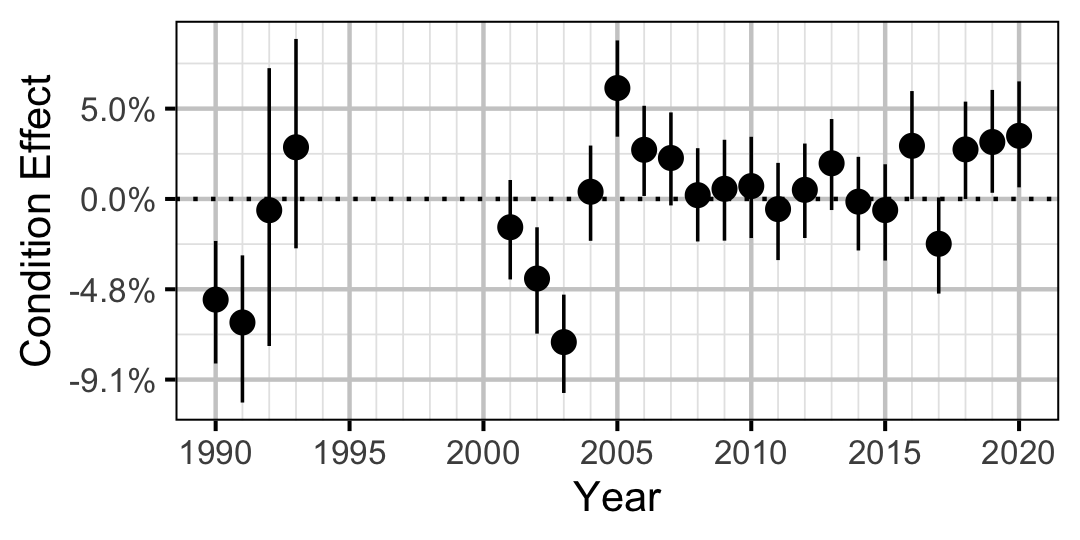Figure 2. Estimated change in condition relative to a typical year for a 200 mm Mountain Whitefish by year (with 95% CRIs).
###### Rainbow Trout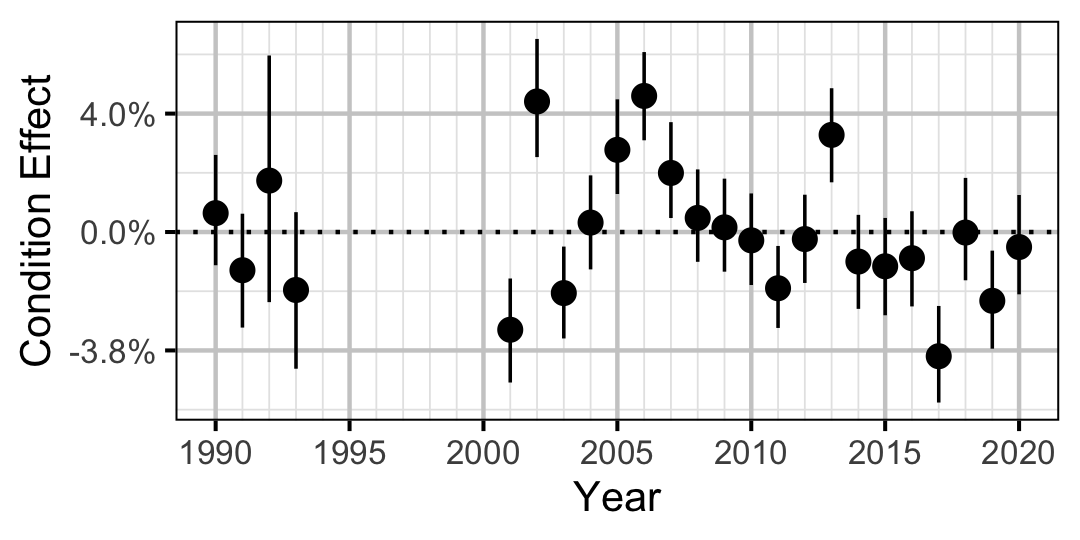Figure 3. Estimated change in condition relative to a typical year for a 250 mm Rainbow Trout by year (with 95% CRIs).
###### Mountain Whitefish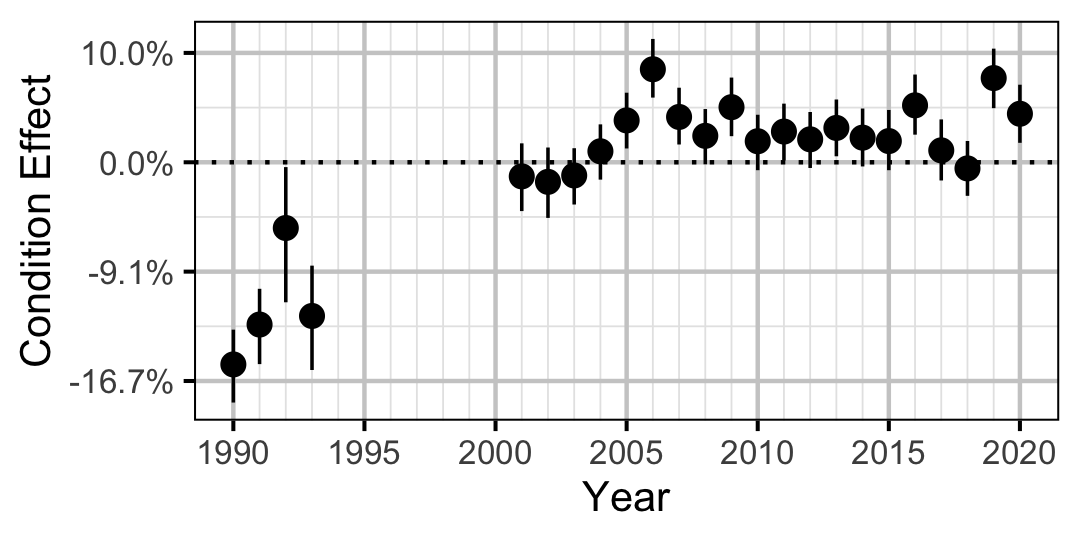Figure 4. Estimated change in condition relative to a typical year for a 350 mm Mountain Whitefish by year (with 95% CRIs).
###### Rainbow Trout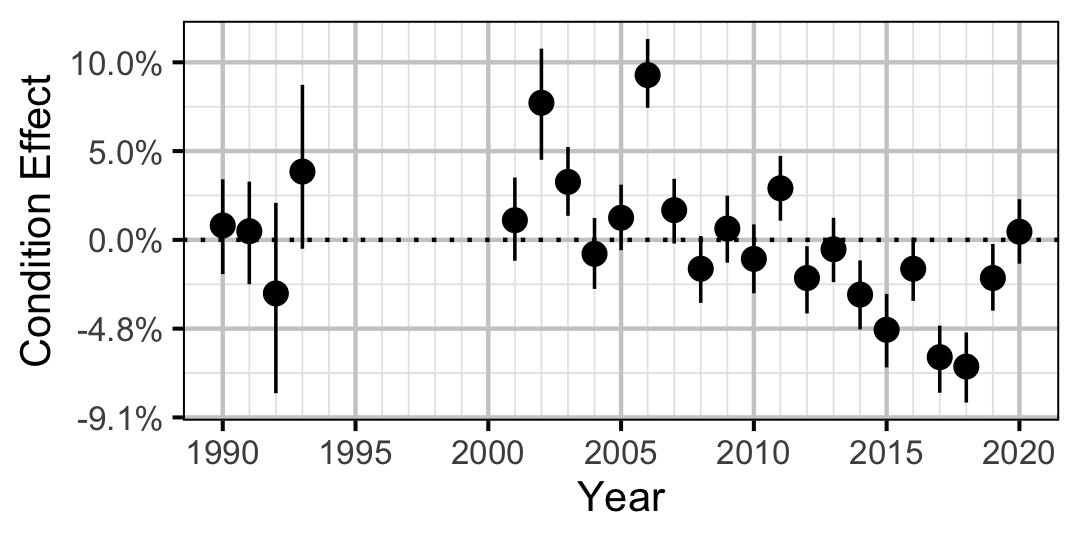Figure 5. Estimated change in condition relative to a typical year for a 500 mm Rainbow Trout by year (with 95% CRIs).
###### Walleye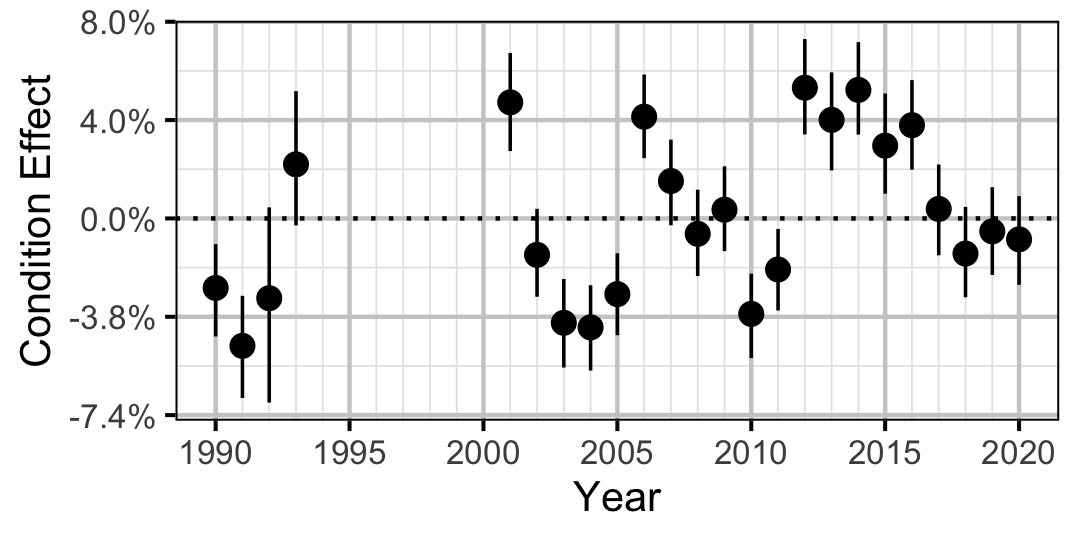Figure 6. Estimated change in condition relative to a typical year for a 400 mm Walleye by year (with 95% CRIs).

#### Growth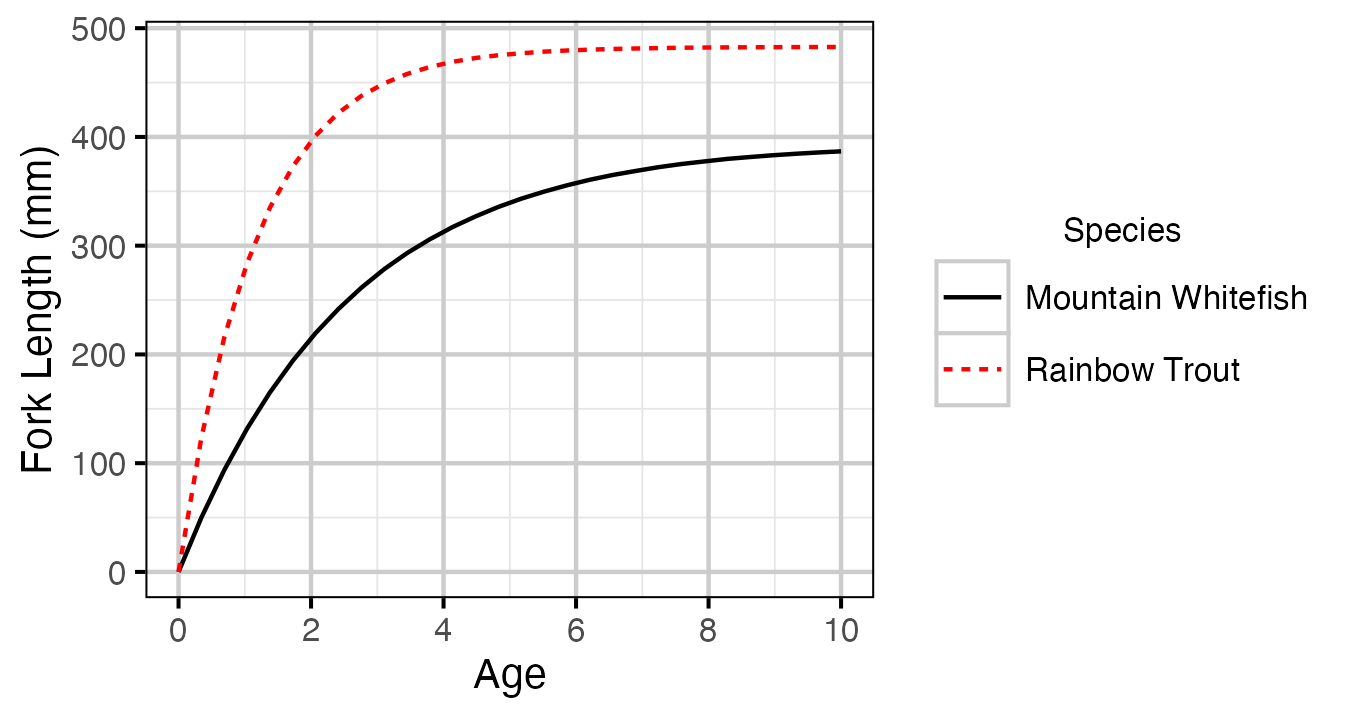Figure 7. Estimated von Bertalanffy growth curve by species. The growth curve for Walleye is not shown because the growth parameters were not representative for the younger age-classes.
##### Mountain Whitefish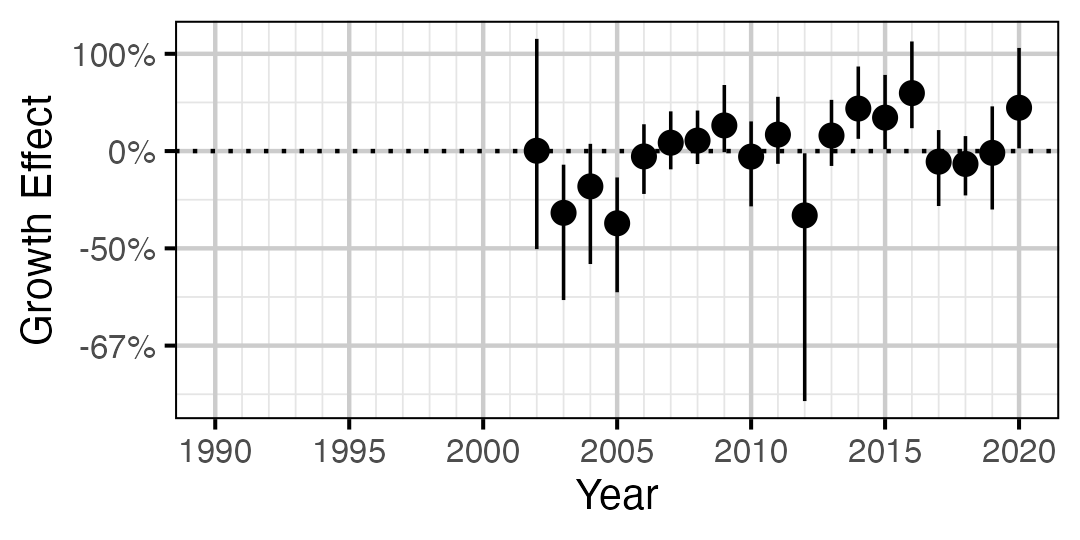Figure 8. Estimated change in von Bertalanffy growth coefficient (k) relative to a typical year by year (with 95% CIs).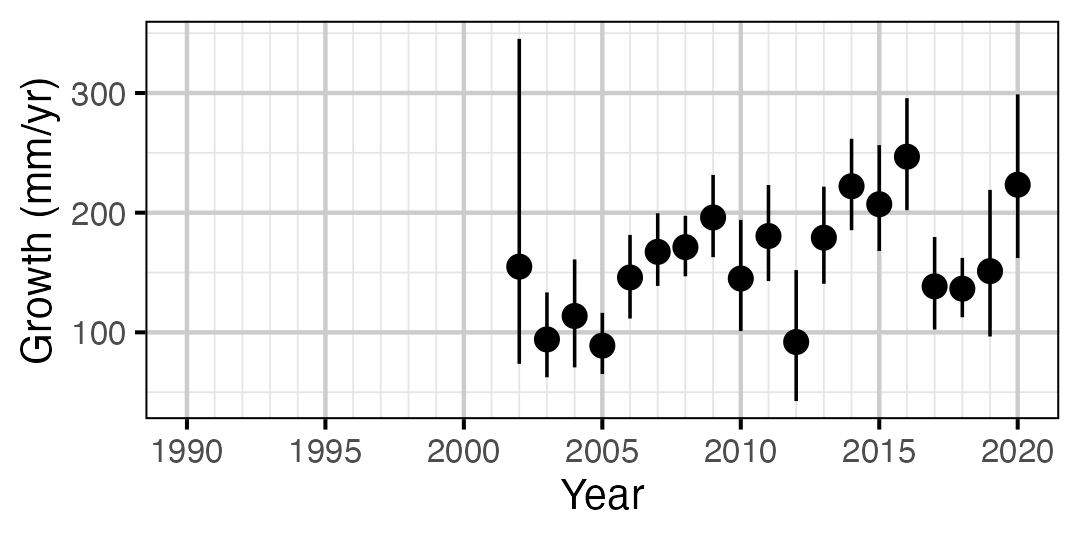Figure 9. Predicted maximum growth by year (with 95% CIs).
##### Rainbow Trout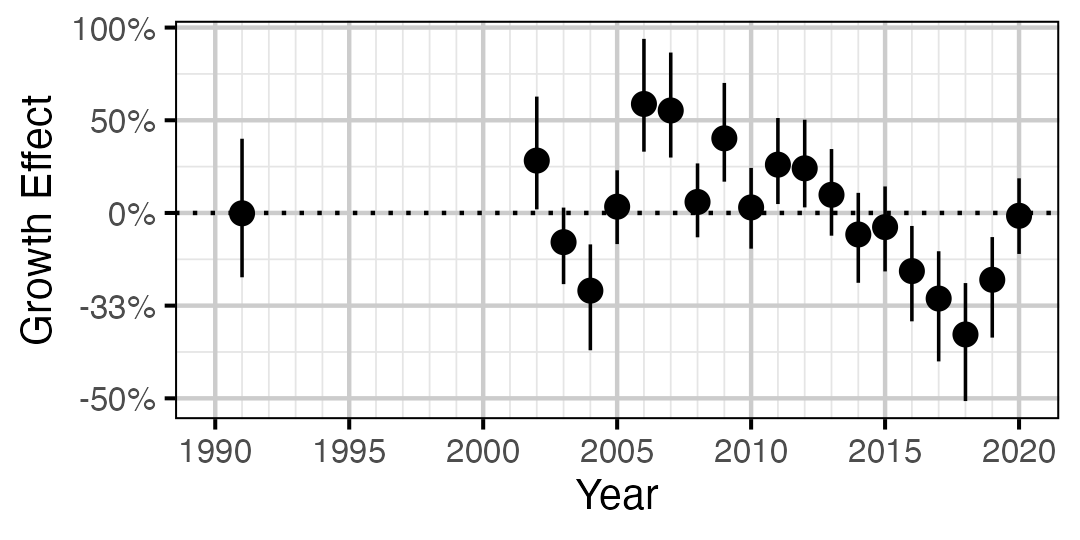Figure 10. Estimated change in von Bertalanffy growth coefficient (k) relative to a typical year by year (with 95% CIs).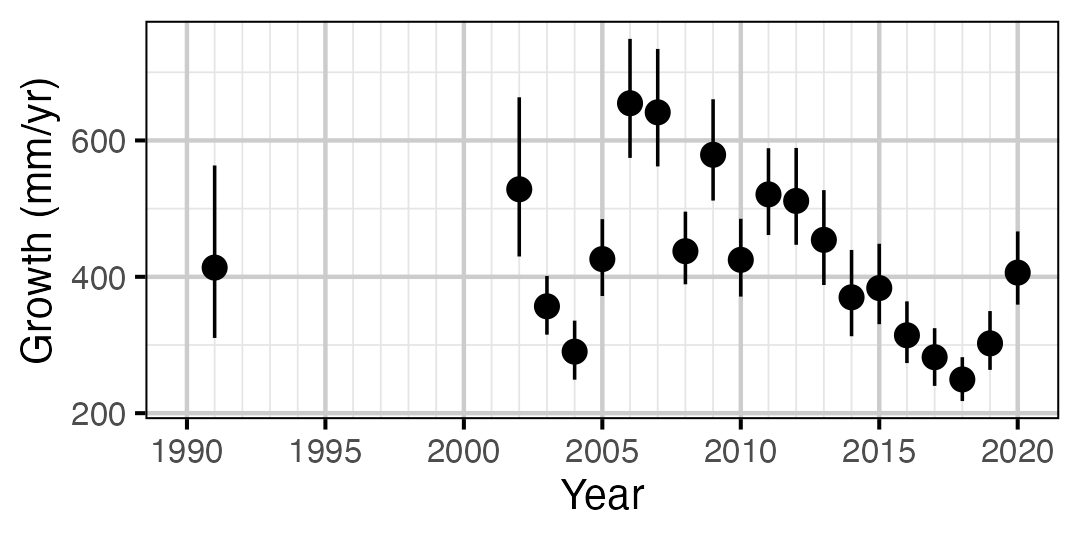Figure 11. Predicted maximum growth by year (with 95% CIs).
##### Walleye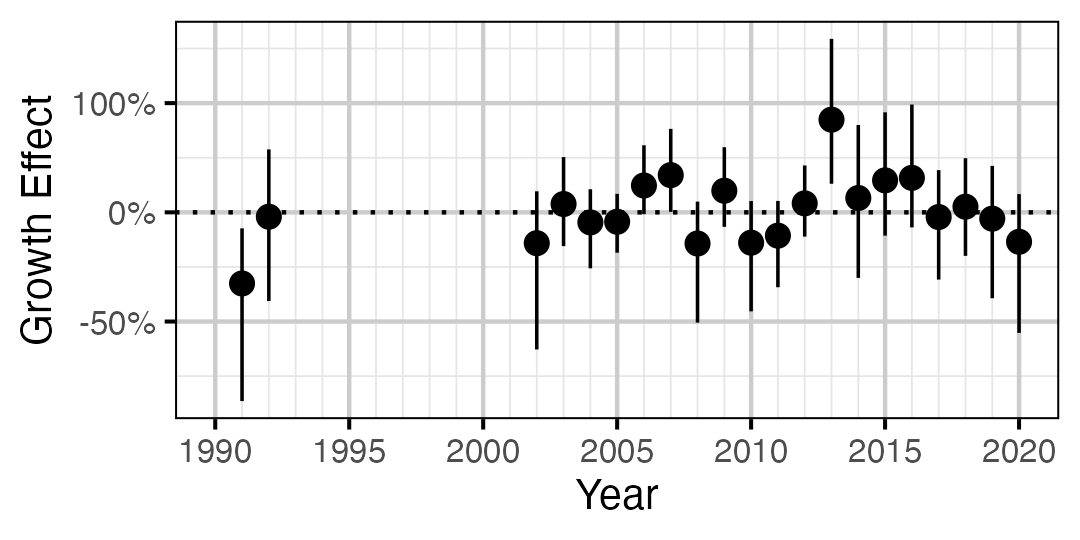Figure 12. Estimated change in von Bertalanffy growth coefficient (k) relative to a typical year by year (with 95% CIs).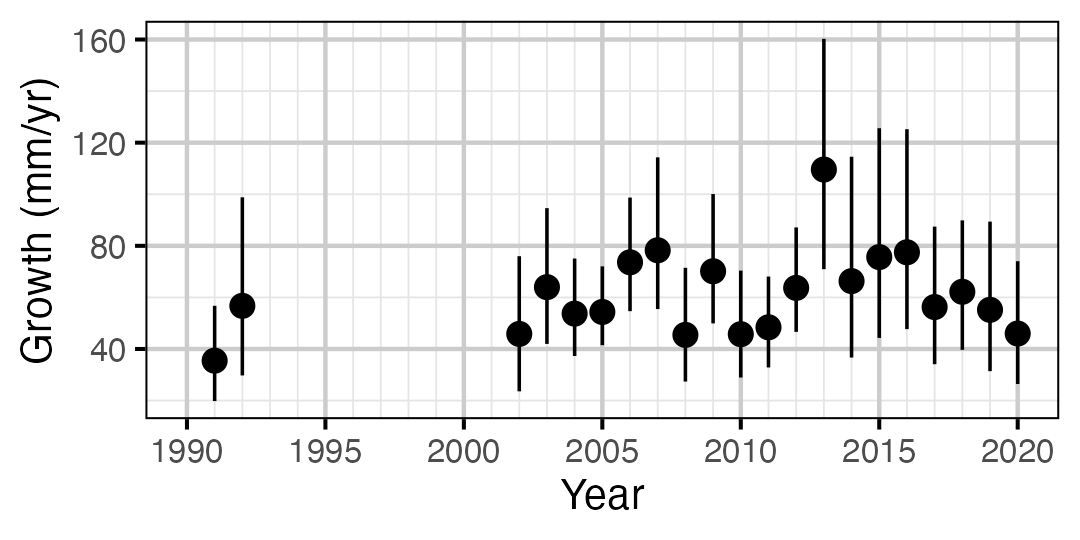Figure 13. Predicted maximum growth by year (with 95% CIs).

#### Movement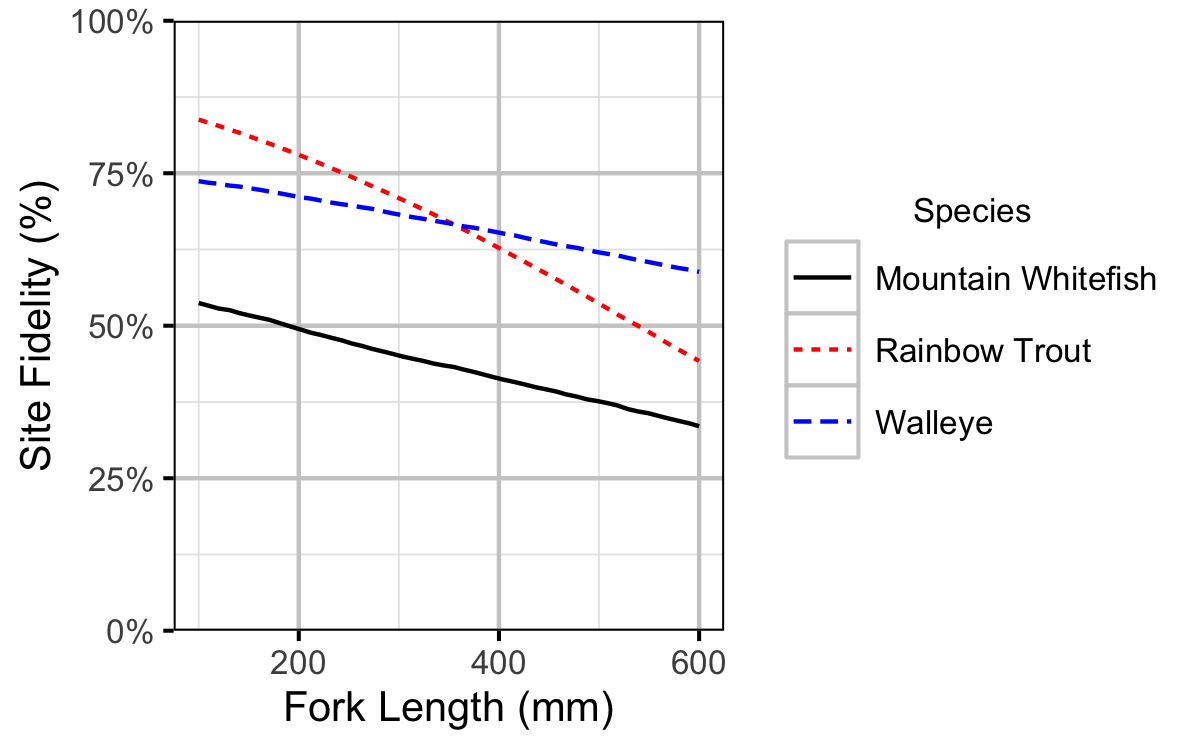Figure 14. Probability of recapture at the same site versus a different site by fish length (with 95% CRIs).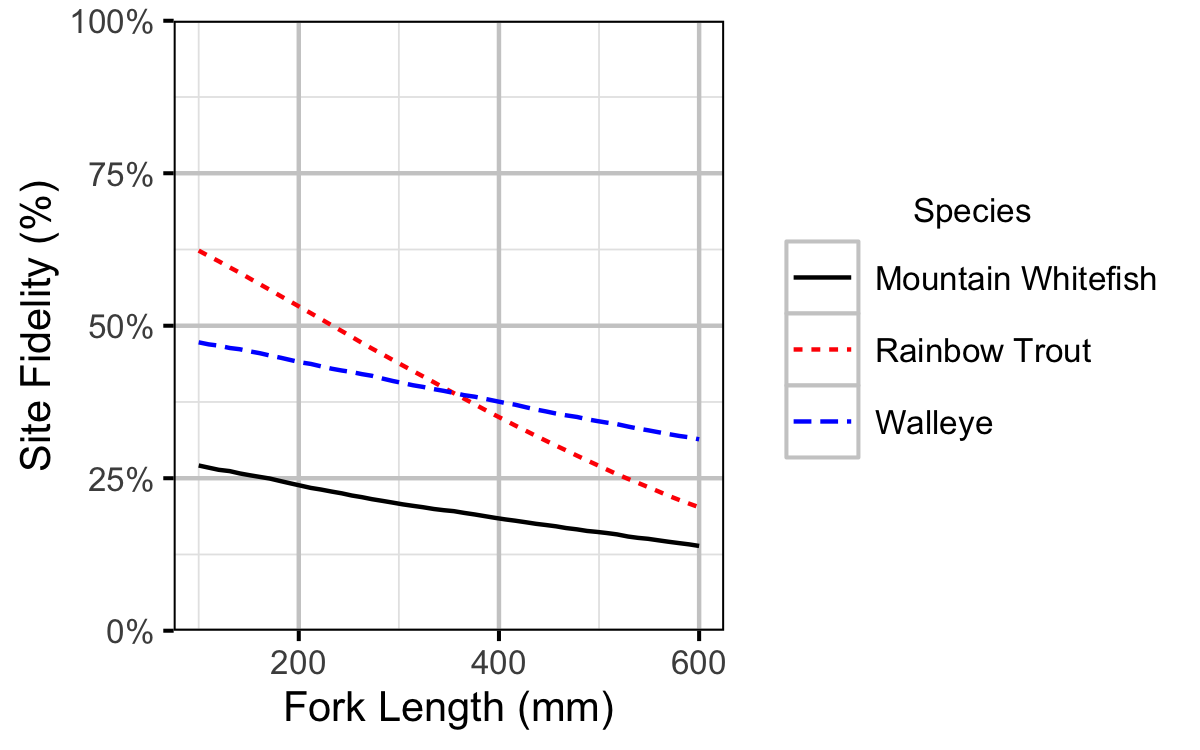Figure 15. Site fidelity by fish length (with 95% CRIs).

#### Observer Length Correction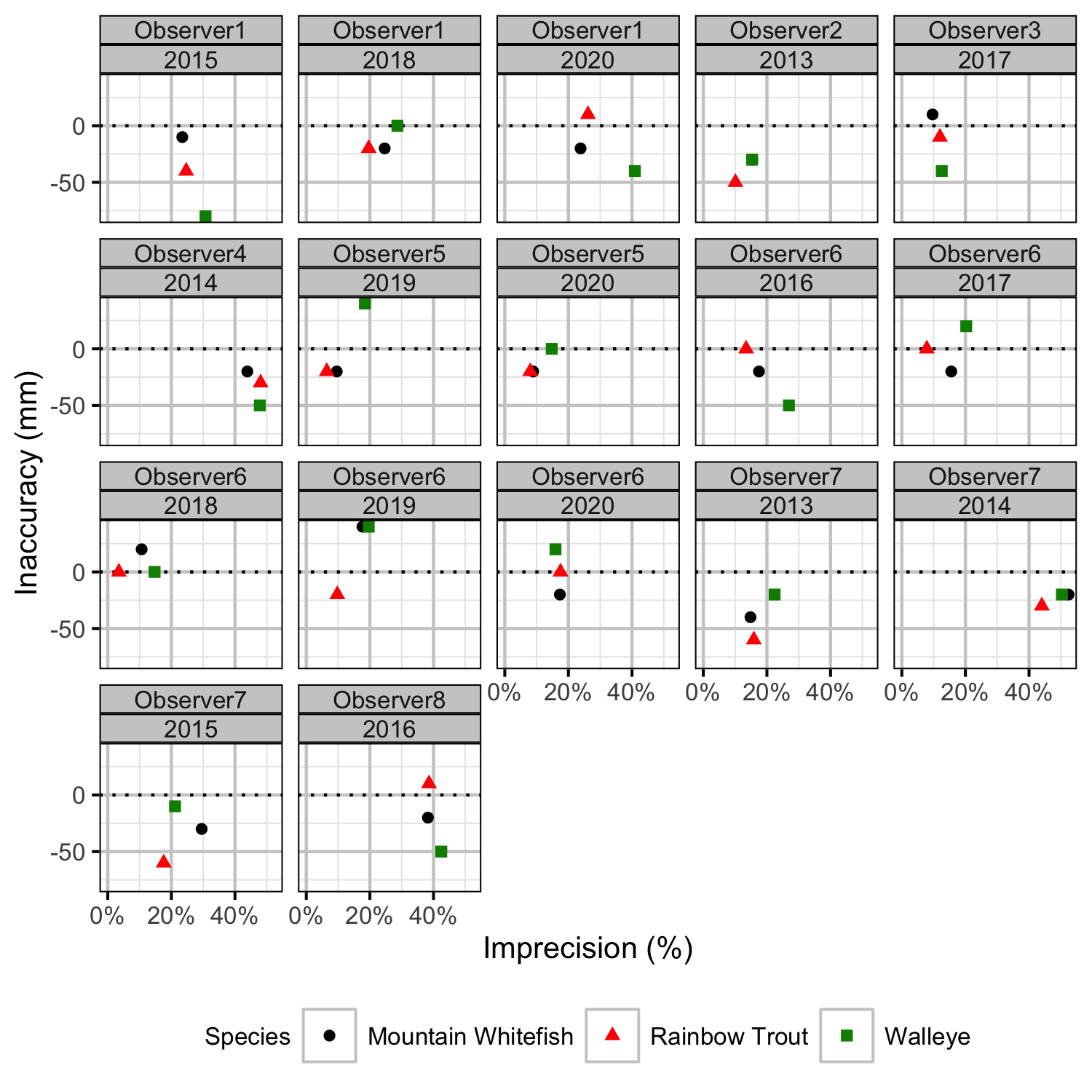Figure 31. Length inaccuracy and imprecision by observer, year and species.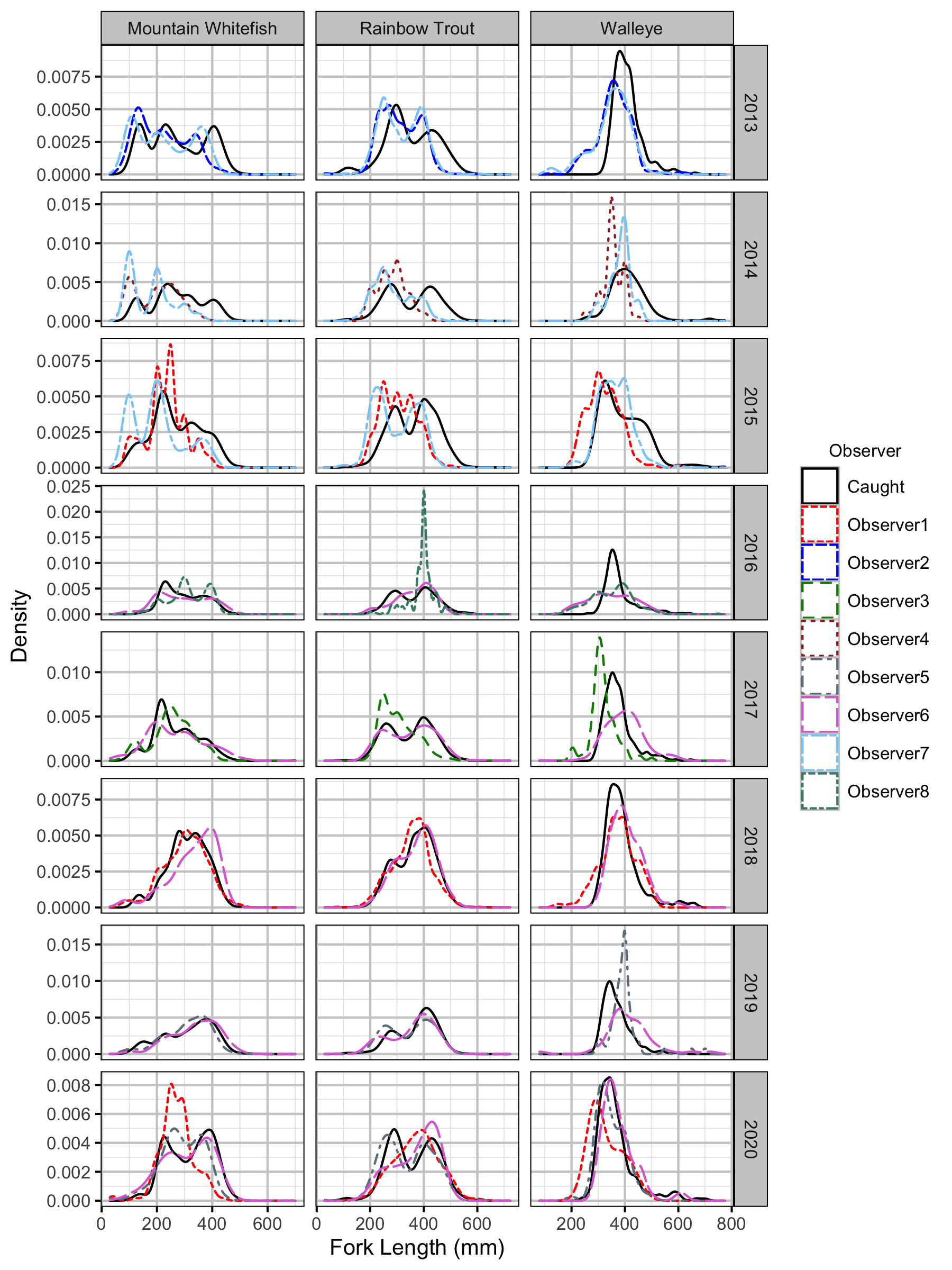Figure 32. Uncorrected length density plots by species, year and observer.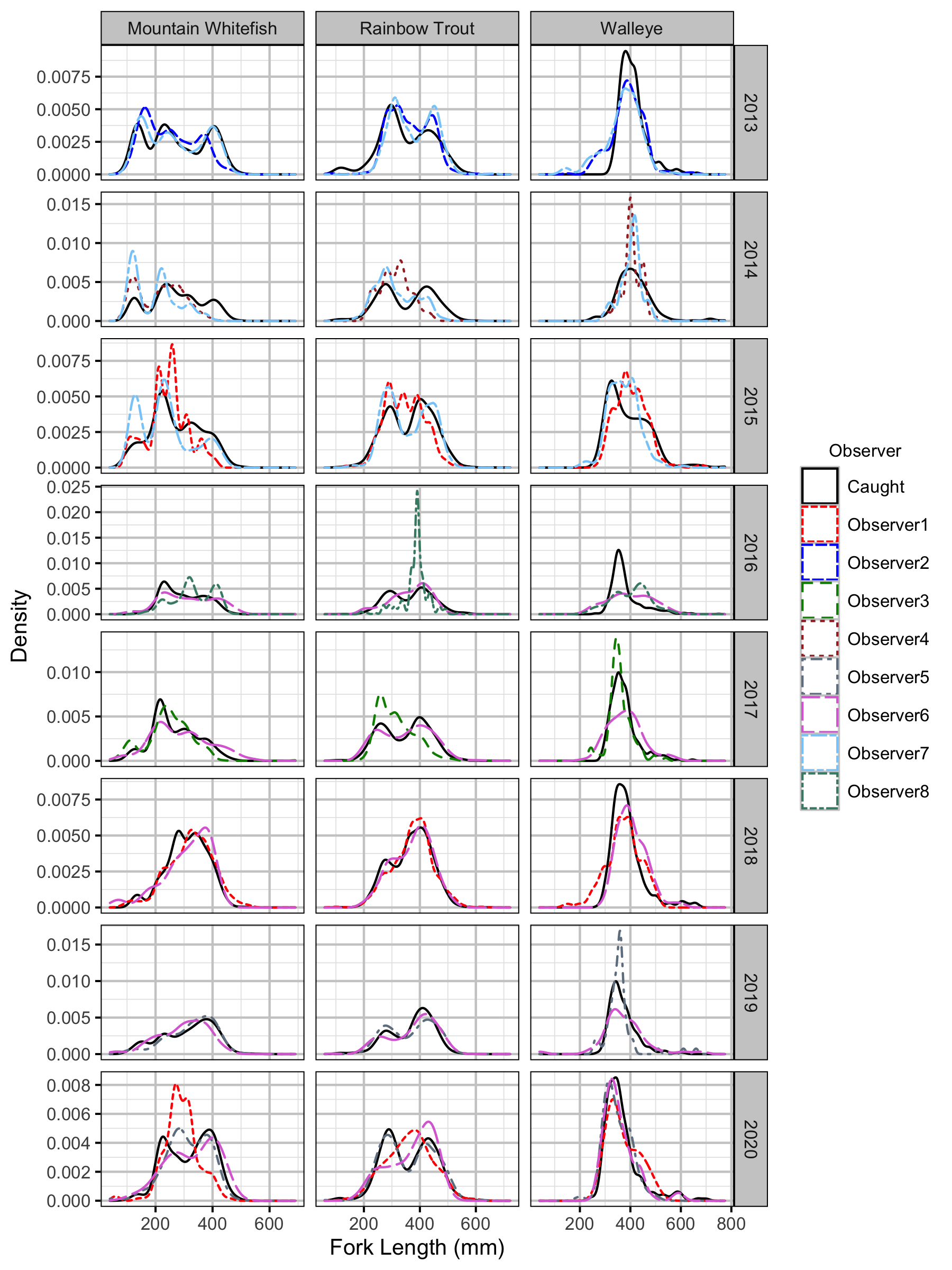Figure 33. Corrected length density plots by species, year and observer.

#### Capture Efficiency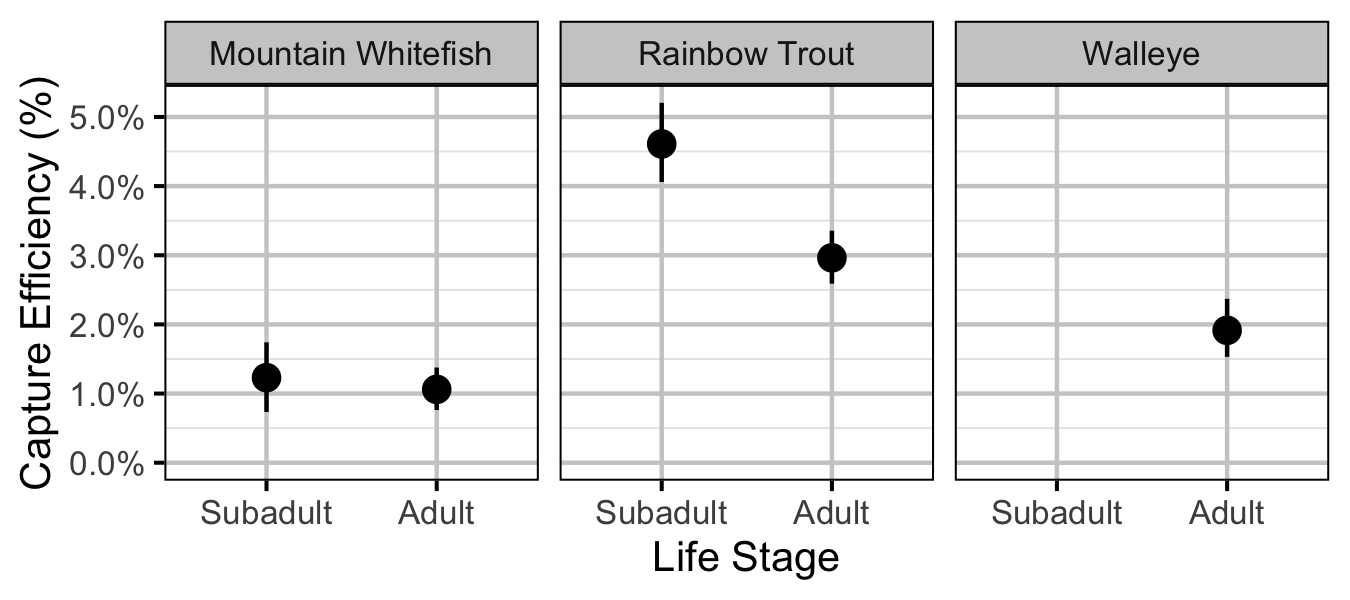Figure 34. Predicted capture efficiency by species and life stage (with 95% CRIs).

#### Abundance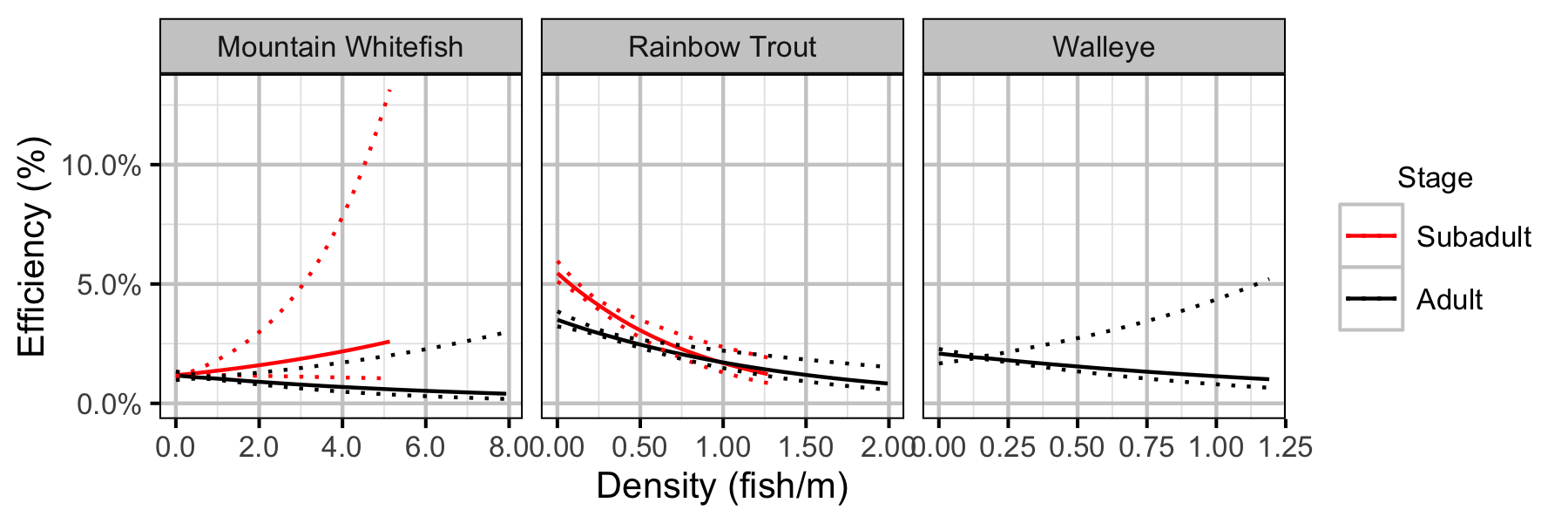Figure 40. Effect of density on capture efficiency by species and stage (with 95% CIs).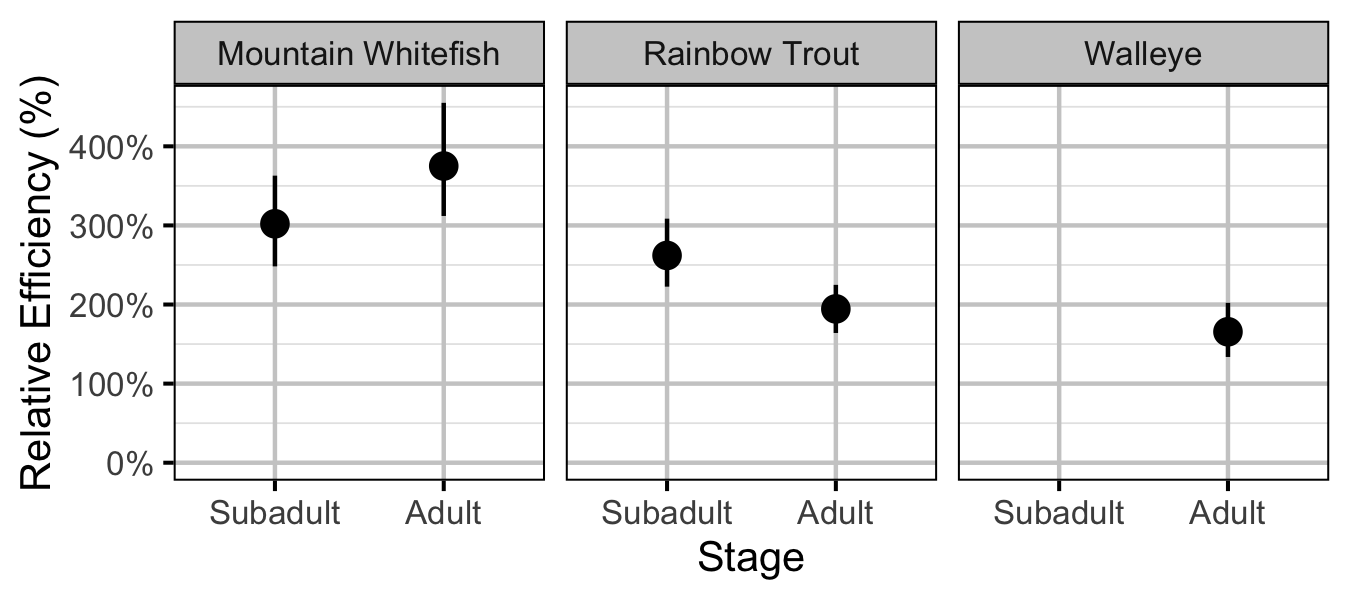Figure 41. Effect of counting (versus capture) on encounter efficiency at typical density by species and stage (with 95% CIs).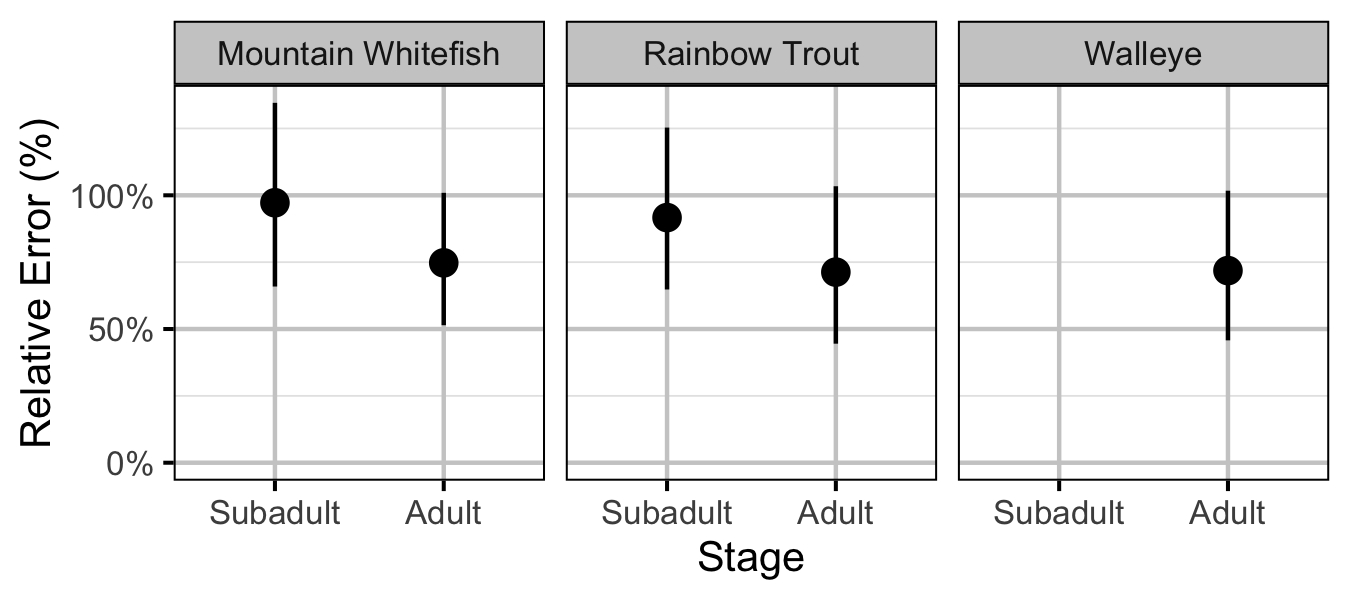Figure 42. Effect of counting (versus capture) on overdispersion by species and stage (with 95% CIs).

#### Fecundity

##### Mountain Whitefish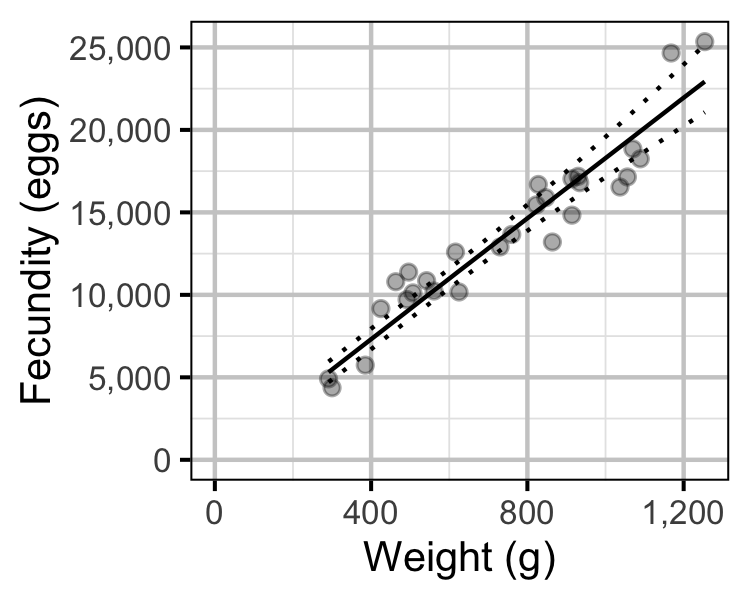Figure 67. The fecundity-weight relationship for Mountain Whitefish (with 95% CRIs). The data are from Boyer et al (2017).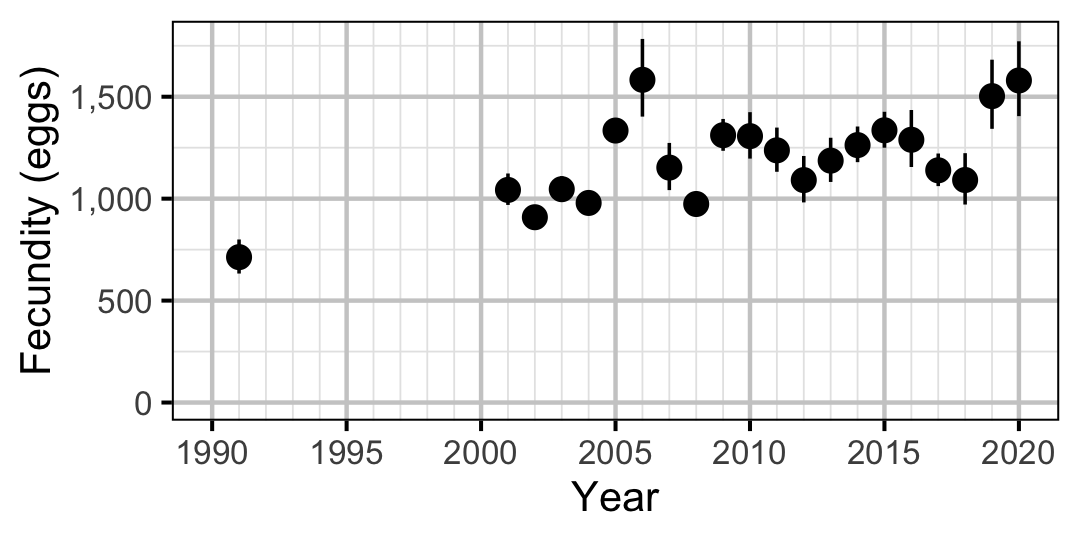Figure 68. Predicted fecundity of an adult female Mountain Whitefish by year (with 95% CIs).

#### Egg Deposition

##### Mountain Whitefish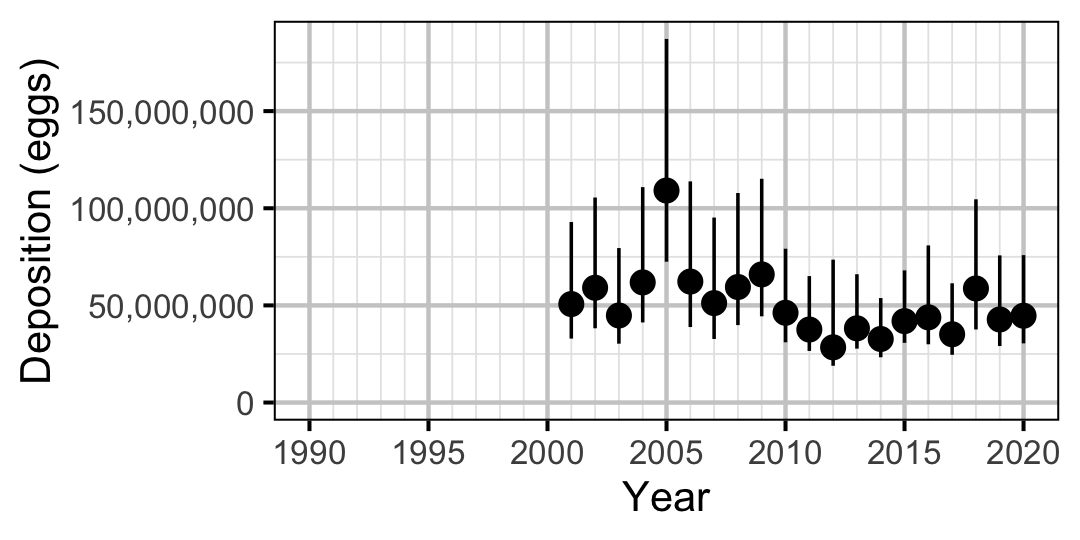Figure 70. Predicted total egg deposition by Mountain Whitefish by year (with 95% CIs).
##### Rainbow Trout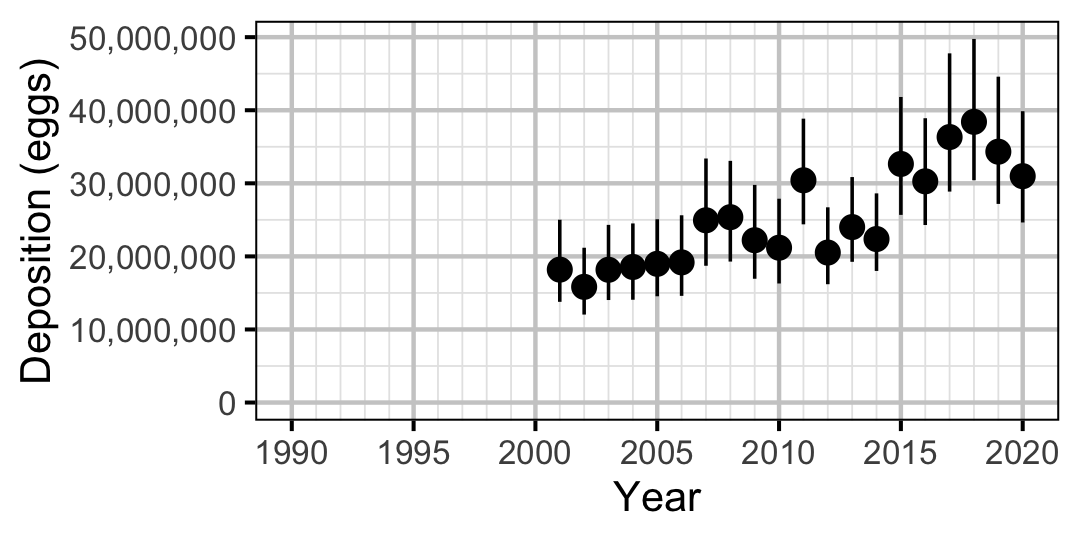Figure 71. Predicted total egg deposition by Rainbow Trout by year (with 95% CIs).

#### Stock-Recruitment

##### Mountain Whitefish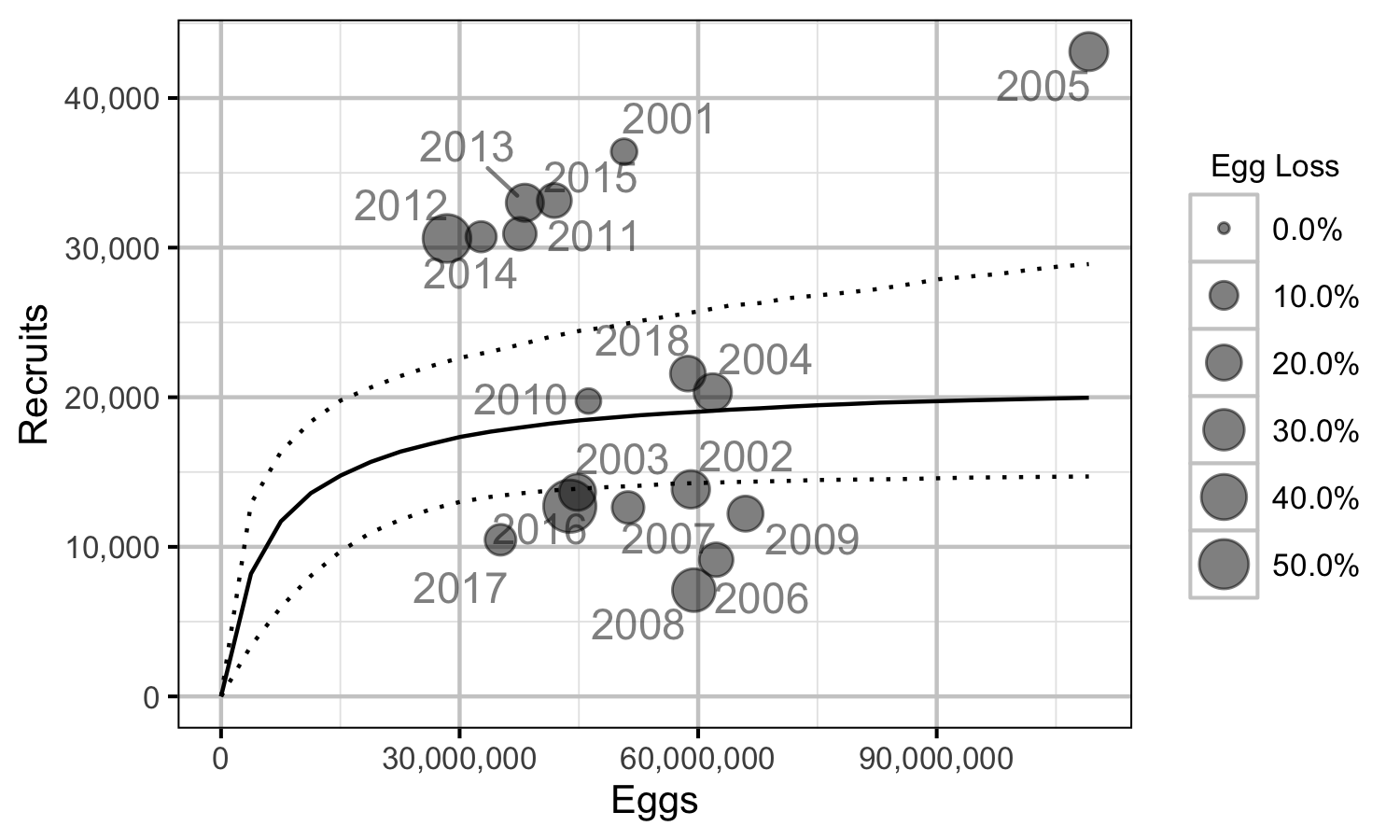Figure 72. Predicted stock-recruitment relationship by spawn year (with 95% CRIs).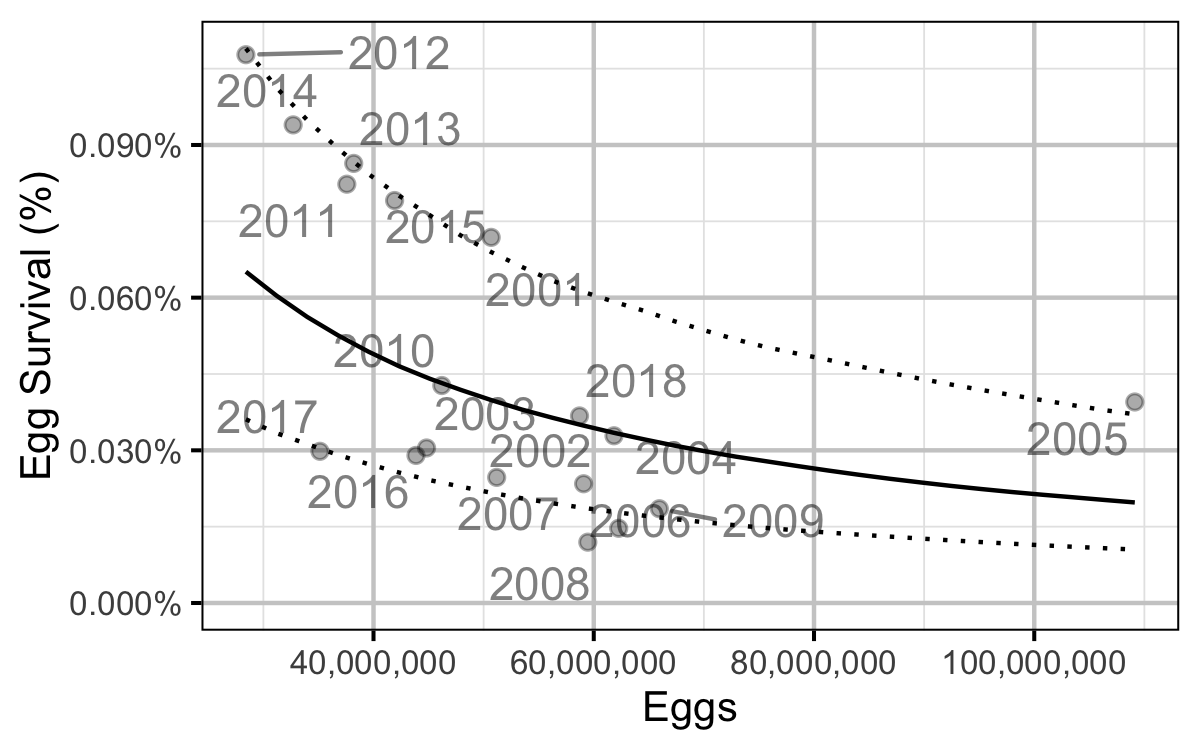Figure 73. Predicted egg to age-1 survival by total egg deposition (with 95% CRIs).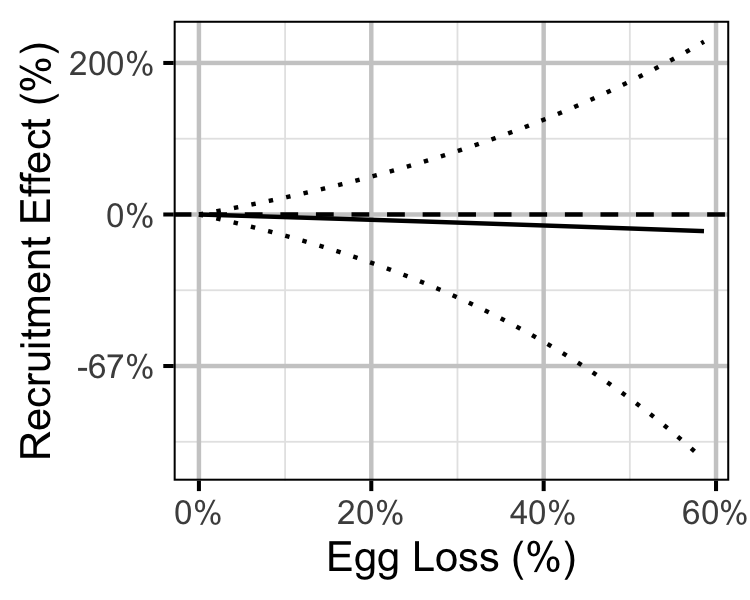Figure 74. Predicted effect of egg loss on the number of age-1 recruits (with 95% CRIs).
##### Rainbow Trout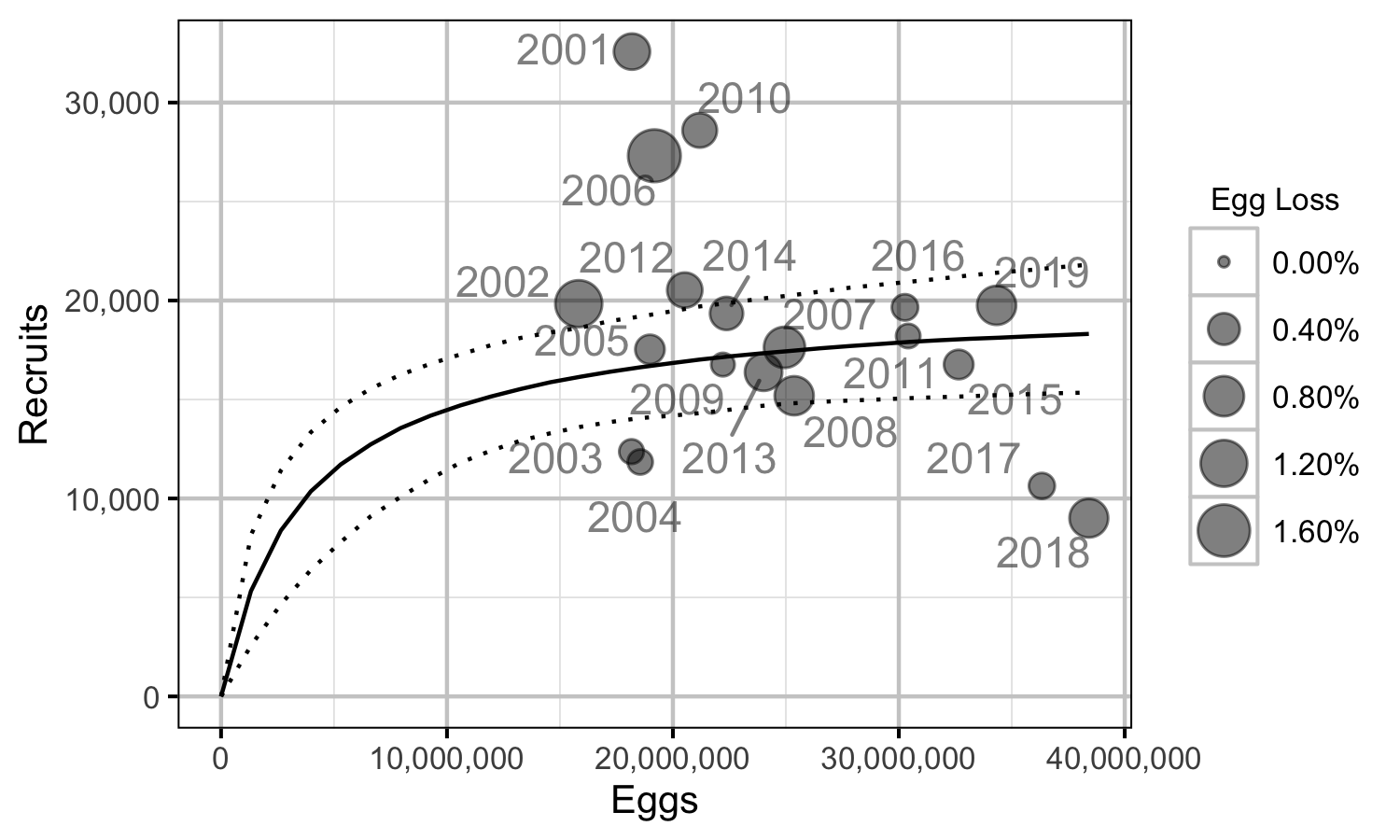Figure 75. Predicted stock-recruitment relationship by spawn year (with 95% CRIs).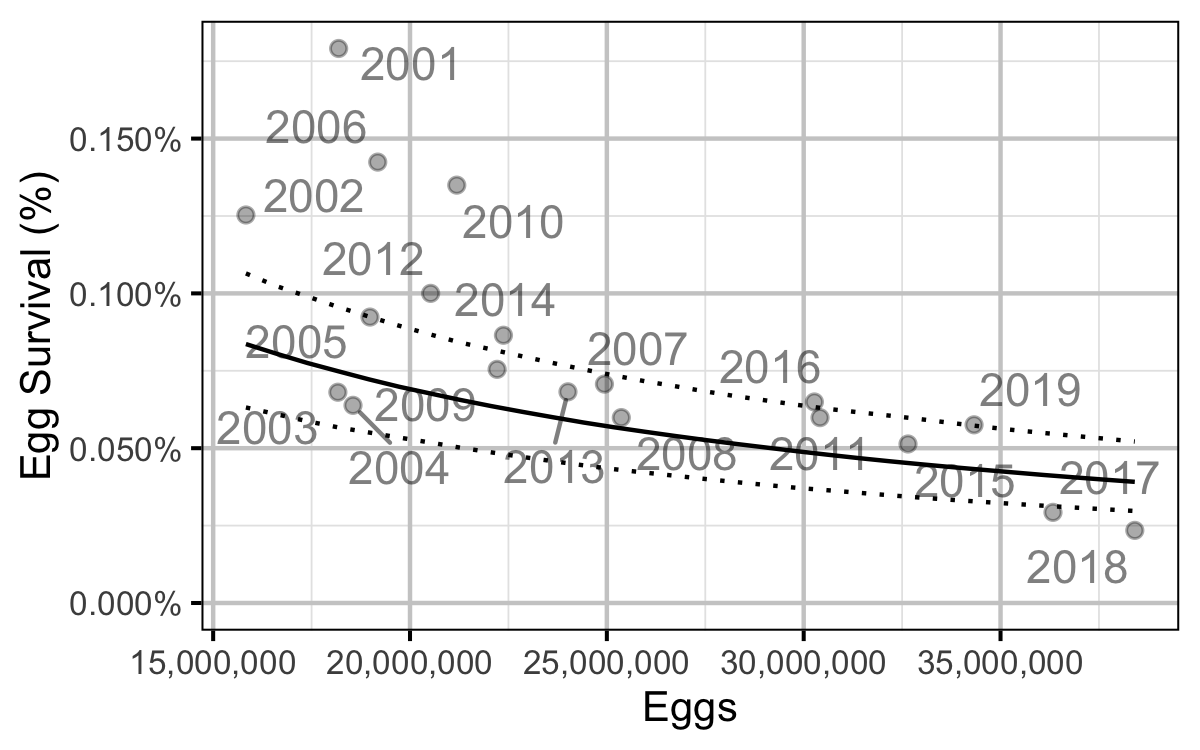Figure 76. Predicted egg to age-1 survival by total egg deposition (with 95% CRIs).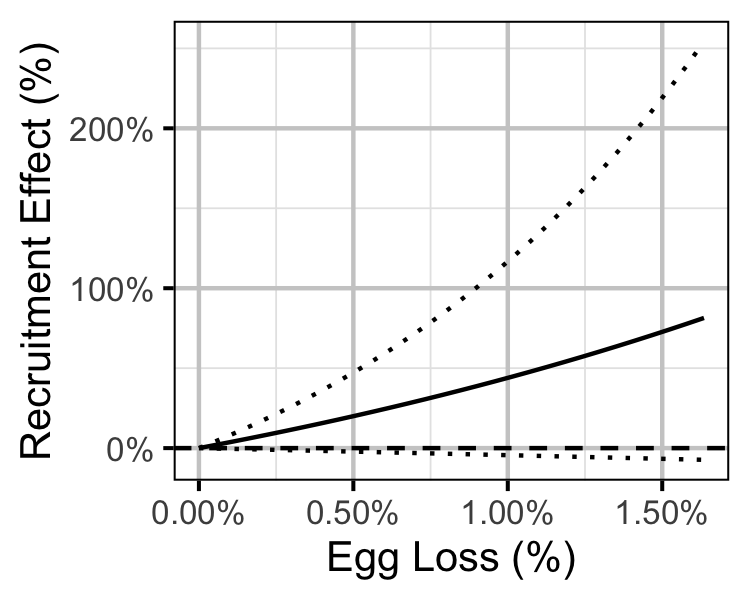Figure 77. Predicted effect of egg loss on the number of age-1 recruits (with 95% CRIs).

#### Age-Ratios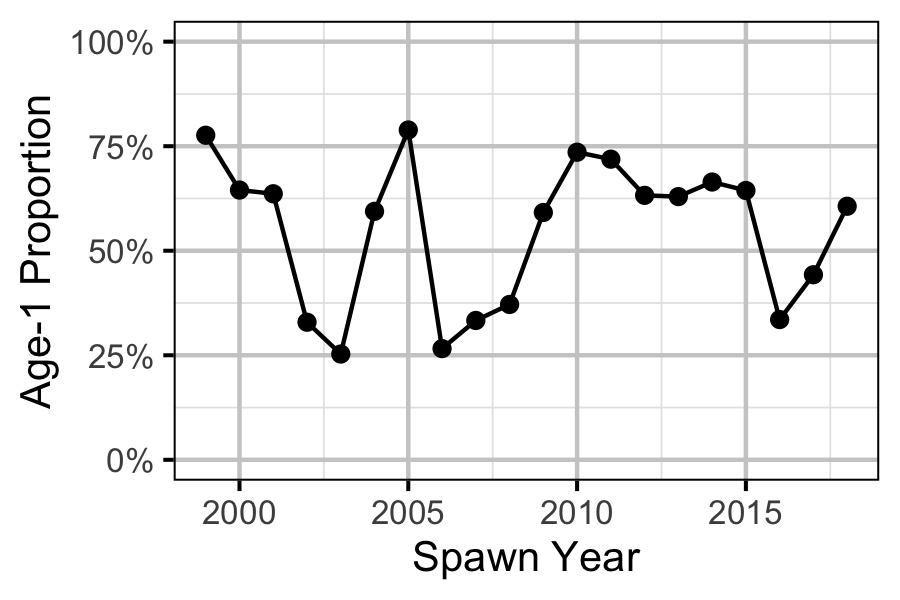Figure 78. Proportion of Age-1 Mountain Whitefish by spawn year.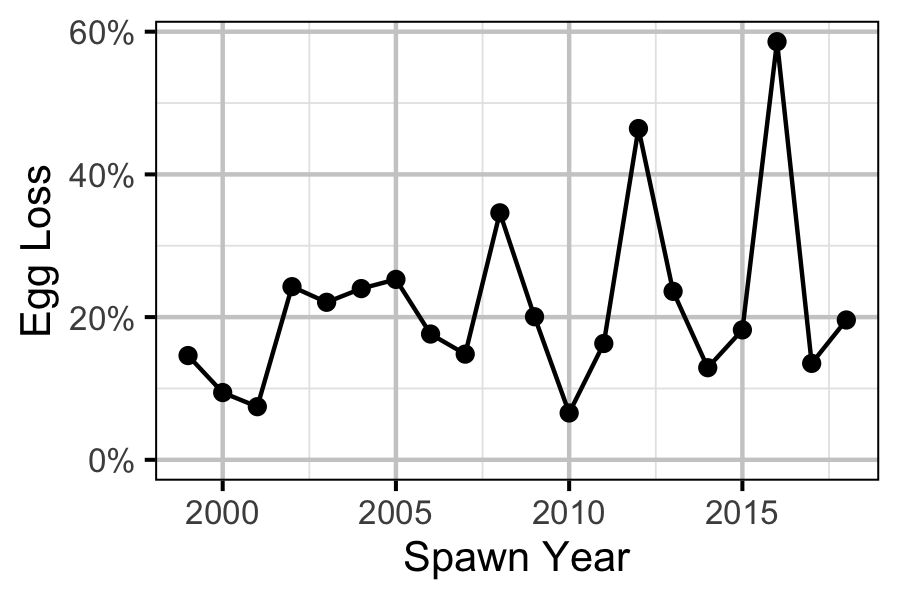Figure 79. Percentage Mountain Whitefish egg loss by spawn year.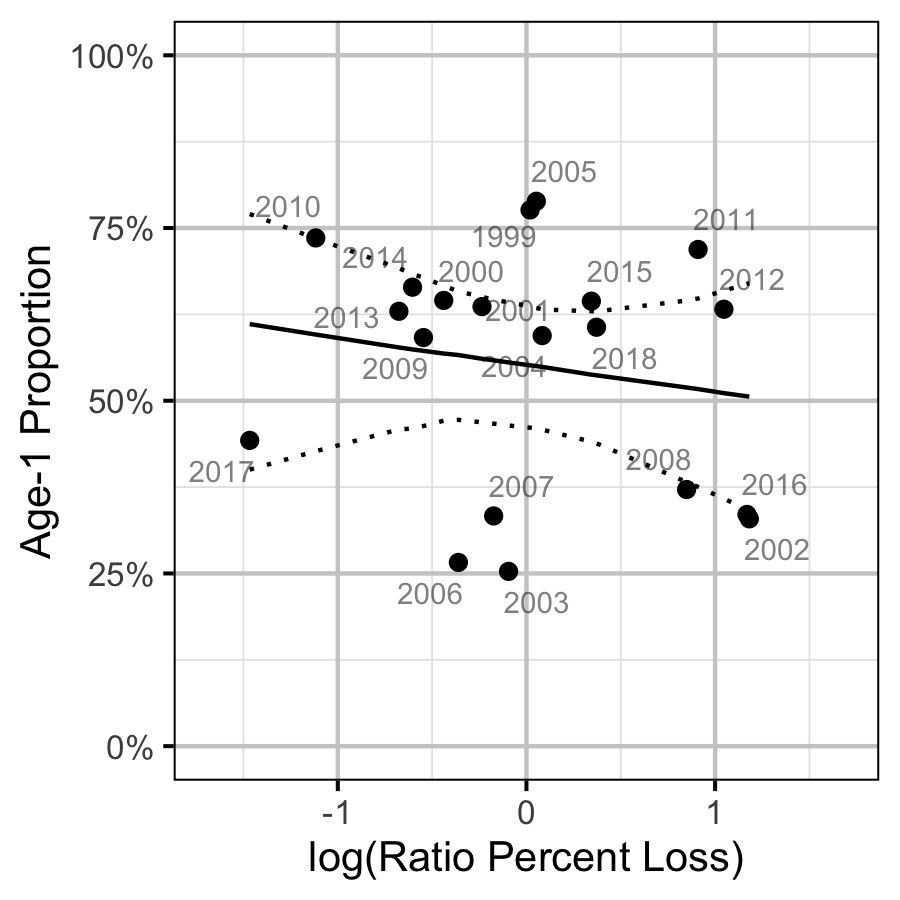Figure 80. Proportion of Age-1 Mountain Whitefish by percentage egg loss ratio, labelled by spawn year. The predicted relationship is indicated by the solid black line (with 95% CRIs).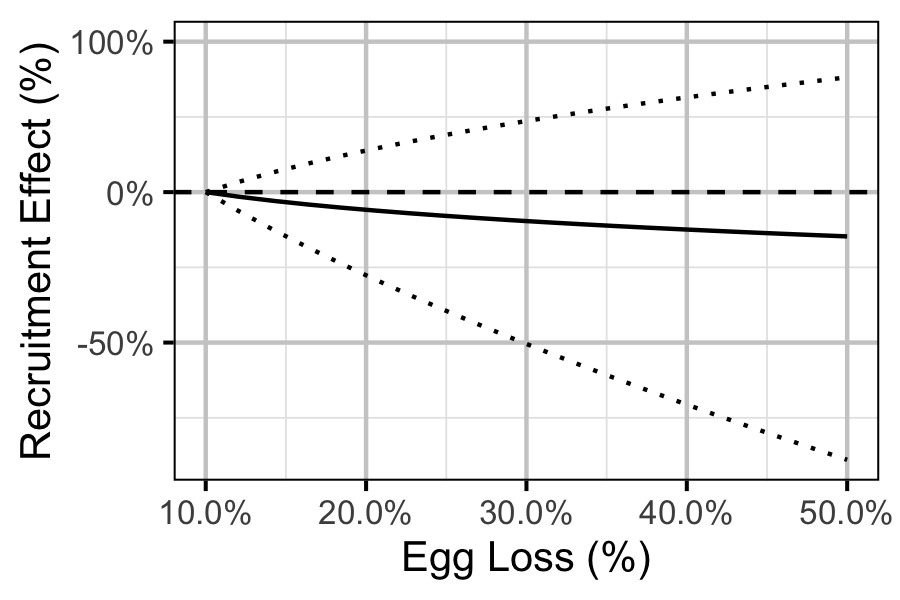Figure 81. Predicted effect of egg loss on the number of age-1 recruits by egg loss relative to 10% egg loss (with 95% CRIs).

## Recommendations

• Develop fecundity vs weight relationship for Mountain Whitefish and Rainbow Trout on the Lower Columbia River.

## Acknowledgements

The organisations and individuals whose contributions have made this analysis report possible include: